SLAS697E March   2010  – November 2016

PRODUCTION DATA.

• PM|64
• KGD|0

## 4 Specifications

### 4.1 Absolute Maximum Ratings(1)

MIN MAX UNIT
Voltage applied at VCC to VSS –0.3 4.1 V
Voltage applied to any pin(2) –0.3 VCC + 0.3 V
Diode current at any device terminal –2 2 mA
Tstg Storage temperature (unprogrammed device(3)) –55 150 °C
Storage temperature (programmed device(3)) –55 150
Stresses beyond those listed under Absolute Maximum Ratings may cause permanent damage to the device. These are stress ratings only, and functional operation of the device at these or any other conditions beyond those indicated under Recommended Operating Conditions is not implied. Exposure to absolute-maximum-rated conditions for extended periods may affect device reliability.
All voltages referenced to VSS. The JTAG fuse-blow voltage, VFB, is allowed to exceed the absolute maximum rating. The voltage is applied to the TEST pin when blowing the JTAG fuse.
Higher temperature may be applied during board soldering process according to the current JEDEC J-STD-020 specification with peak reflow temperatures not higher than classified on the device label on the shipping boxes or reels.

### 4.2 ESD Ratings

MAX UNIT
V(ESD) Electrostatic discharge Human-body model (HBM), per ANSI/ESDA/JEDEC JS001(1) ±4000 V
Charged-device model (CDM), per JESD22-C101(2) ±750
JEDEC document JEP155 states that 500-V HBM allows safe manufacturing with a standard ESD control process.
JEDEC document JEP157 states that 250-V CDM allows safe manufacturing with a standard ESD control process.

### 4.3 Recommended Operating Conditions(1)(3)

MIN NOM MAX UNIT
VCC Supply voltage during program execution AVCC = DVCC = VCC(2) 1.8 3.6 V
Supply voltage during flash memory programming 2.2 3.6
VSS Supply voltage AVSS = DVSS = VSS 0 V
TA Operating free-air temperature range –55 150 °C
Processor frequency ƒSYSTEM
(Maximum MCLK frequency)(1)(3)
(see Figure 4-1)
VCC = 2.2 V, duty cycle = 50% ±10% DC 10 MHz
VCC = 2.7 V, duty cycle = 50% ±10% DC 12
VCC ≥ 3.3 V, duty cycle = 50% ±10% DC 16
The MSP430 CPU is clocked directly with MCLK. Both the high and low phase of MCLK must not exceed the pulse width of the specified maximum frequency.
It is recommended to power AVCC and DVCC from the same source. A maximum difference of 0.3 V between AVCC and DVCC can be tolerated during power-up.
Modules might have a different maximum input clock specification. Refer to the specification of the respective module in this data sheet.NOTE:

Minimum processor frequency is defined by system clock. Flash program or erase operations require a minimum VCC of 2.2 V.
Figure 4-1 Operating AreaWirebond Life = Time at temperature with or without bias.
Electromigration Fail Mode = Time at temperature with bias.
Silicon operating life design goal is 10 years at 105˚C junction temperature (does not include package interconnect life).
The predicted operating lifetime vs. junction temperature is based on reliability modeling and available qualification data.
Figure 4-2 Device Life Curve

### 4.4 Thermal Information

THERMAL METRIC(1) MSP430F2619S-HT UNIT
PM (QFP)
64 PINS
RθJA Junction-to-ambient thermal resistance 48.7 °C/W
RθJC(top) Junction-to-case (top) thermal resistance 9.9 °C/W
RθJB Junction-to-board thermal resistance 22.4 °C/W
ψJT Junction-to-top characterization parameter 0.4 °C/W
ψJB Junction-to-board characterization parameter 21.9 °C/W

### 4.5 Active-Mode Supply Current Into AVCC Excluding External Current – Electrical Characteristics(1)(2)

over recommended ranges of supply voltage and operating free-air temperature (unless otherwise noted)
PARAMETER TEST CONDITIONS MIN TYP MAX UNIT
IAM, 1MHz Active-mode (AM) current (1 MHz) ƒDCO = ƒMCLK = ƒSMCLK = 1 MHz,
ƒACLK = 32,768 Hz,
Program executes in flash,
BCSCTL1 = CALBC1_1 MHZ,
DCOCTL = CALDCO_1 MHZ,
CPUOFF = 0, SCG0 = 0,
SCG1 = 0, OSCOFF = 0
TA = –55°C to 85°C,
VCC = 2.2 V
365 395 μA
TA = 105°C, VCC = 2.2 V 375 420
TA = 150°C, VCC = 2.2 V 640
TA = –55°C to 85°C,
VCC = 3 V
515 560
TA = 105°C, VCC = 3 V 525 595
TA = 150°C, VCC = 3 V 700
IAM, 1MHz Active-mode (AM) current (1 MHz) ƒDCO = ƒMCLK = ƒSMCLK = 1 MHz,
ƒACLK = 32,768 Hz,
Program executes in RAM,
BCSCTL1 = CALBC1_1 MHZ,
DCOCTL = CALDCO_1 MHZ,
CPUOFF = 0, SCG0 = 0,
SCG1 = 0, OSCOFF = 0
TA = –55°C to 85°C, VCC = 2.2 V 330 370 μA
TA = 105°C, VCC = 2.2 V 340 390
TA = 150°C, VCC = 2.2 V 660
TA = –55°C to 85°C,
VCC = 3 V
460 495
TA = 105°C, VCC = 3 V 470 520
TA = 150°C, VCC = 3 V 710
IAM, 4kHz Active-mode (AM) current (4 kHz) ƒMCLK = ƒSMCLK = ƒACLK = 32,768 Hz/8
= 4,096 Hz, ƒDCO = 0 Hz,
Program executes in flash,
SELMx = 11, SELS = 1,
DIVMx = DIVSx = DIVAx = 11,
CPUOFF = 0, SCG0 = 1,
SCG1 = 0, OSCOFF = 0
TA = –55°C to 85°C,
VCC = 2.2 V
2.1 9 μA
TA = 105°C, VCC = 2.2 V 15 31
TA = –55°C to 85°C,
VCC = 3 V
3 11
TA = 105°C, VCC = 3 V 19 32
IAM, 100kHz Active-mode (AM) current (100 kHz) ƒMCLK = ƒSMCLK = ƒDCO(0, 0) ≉ 100 kHz,
ƒACLK = 0 Hz, Program executes in flash,
RSELx = 0, DCOx = 0, CPUOFF = 0,
SCG0 = 0, SCG1 = 0, OSCOFF = 1
TA = –55°C to 85°C,
VCC = 2.2 V
67 86 μA
TA = 105°C, VCC = 2.2 V 80 99
TA = 150°C, VCC = 2.2 V 190
TA = –55°C to 85°C, VCC = 3 V 84 107
TA = 105°C, VCC = 3 V 99 128
TA = 150°C, VCC = 3 V 240
All inputs are tied to 0 V or VCC. Outputs do not source or sink any current.
The currents are characterized with a Micro Crystal CC4V-T1A SMD crystal with a load capacitance of 9 pF. The internal and external load capacitance is chosen to closely match the required 9 pF.

### 4.6 Typical Characteristics – Active-Mode Supply Current (Into DVCC + AVCC)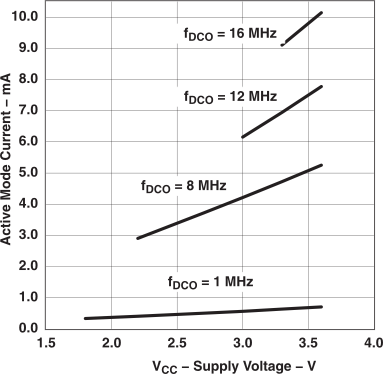Figure 4-3 Active-Mode Current vs VCC, TA = 25°C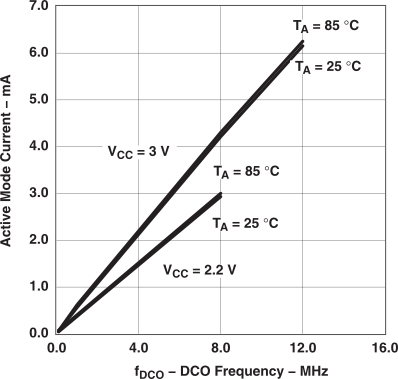Figure 4-4 Active-Mode Current vs DCO Frequency

### 4.7 Active-Mode Current vs DCO Frequency

over recommended ranges of supply voltage and operating free-air temperature (unless otherwise noted)
PARAMETER TEST CONDITIONS MIN TYP MAX UNIT
Active-mode current supply ƒSMCLK = ƒDCO = 1 MHz TA = –55°C, VCC = 2.2 V 0.35 mA
TA = –40°C, VCC = 2.2 V 0.30
TA = 25°C, VCC = 2.2 V 0.36
TA = 125°C, VCC = 2.2 V 0.38
TA = 150°C, VCC = 2.2 V 0.42
TA = –55°C, VCC = 3 V 0.50
TA = –40°C, VCC = 3 V 0.49
TA = 25°C, VCC = 3 V 0.51
TA = 125°C, VCC = 3 V 0.55
TA = 150°C, VCC = 3 V 0.60
Active-mode current supply ƒSMCLK = ƒDCO = 12 MHz TA = –55°C, VCC = 2.2 V 3.71 mA
TA = –40°C, VCC = 2.2 V 3.73
TA = 25°C, VCC = 2.2 V 3.79
TA = 125°C, VCC = 2.2 V 4.45
TA = 150°C, VCC = 2.2 V 4.60
TA = –55°C, VCC = 3 V 5.47
TA = –40°C, VCC = 3 V 5.49
TA = 25°C, VCC = 3 V 5.54
TA = 125°C, VCC = 3 V 5.68
TA = 150°C, VCC = 3 V 5.77
Active-mode current supply ƒSMCLK = ƒDCO = 16 MHz TA = –55°C, VCC = 2.2 V 5.46 mA
TA = –40°C, VCC = 2.2 V 5.58
TA = 25°C, VCC = 2.2 V 5.89
TA = 125°C, VCC = 2.2 V 6.03
TA = 150°C, VCC = 2.2 V 6.20
TA = –55°C, VCC = 3 V 7.14
TA = –40°C, VCC = 3 V 7.14
TA = 25°C, VCC = 3 V 7.21
TA = 125°C, VCC = 3 V 7.429
TA = 150°C, VCC = 3 V 7.54

### 4.8 Low-Power-Mode Supply Currents Into AVCC Excluding External Current – Electrical Characteristics(1)(2)

over recommended ranges of supply voltage and operating free-air temperature (unless otherwise noted)
PARAMETER TEST CONDITIONS MIN TYP MAX UNIT
ILPM0, 1MHz Low-power mode 0 (LPM0) current(3) ƒMCLK = 0 MHz,
ƒSMCLK = ƒDCO = 1 MHz,
ƒACLK = 32,768 Hz,
BCSCTL1 = CALBC1_1 MHZ,
DCOCTL = CALDCO_1 MHZ,
CPUOFF = 1, SCG0 = 0, SCG1 = 0, OSCOFF = 0
TA = –55°C to 85°C,
VCC = 2.2 V
68 83 μA
TA = 105°C, VCC = 2.2 V 83 98
TA = 150°C, VCC = 2.2 V 210
TA = –55°C to 85°C,
VCC = 3 V
87 105
TA = 105°C, VCC = 3 V 100 125
TA = 150°C, VCC = 3 V 240
ILPM0, 100kHz Low-power mode 0 (LPM0) current(3) ƒMCLK = 0 MHz,
ƒSMCLK = ƒDCO(0, 0) ≉ 100 kHz,
ƒACLK = 0 Hz,
RSELx = 0, DCOx = 0,
CPUOFF = 1, SCG0 = 0, SCG1 = 0, OSCOFF = 1
TA = –55°C to 85°C,
VCC = 2.2 V
37 49 μA
TA = 105°C, VCC = 2.2 V 50 62
TA = 150°C, VCC = 2.2 V 160
TA = –55°C to 85°C,
VCC = 3 V
40 55
TA = 105°C, VCC = 3 V 57 73
TA = 150°C, VCC = 3 V 185
ILPM2 Low-power mode 2 (LPM2) current(4) ƒMCLK = ƒSMCLK = 0 MHz, ƒDCO = 1 MHz,
ƒACLK = 32,768 Hz,
BCSCTL1 = CALBC1_1 MHZ,
DCOCTL = CALDCO_1 MHZ,
CPUOFF = 1, SCG0 = 0, SCG1 = 1, OSCOFF = 0
TA = –55°C to 85°C,
VCC = 2.2 V
23 33 μA
TA = 105°C, VCC = 2.2 V 35 46
TA = 150°C, VCC = 2.2 V 148
TA = –55°C to 85°C,
VCC = 3 V
25 36
TA = 105°C, VCC = 3 V 40 55
TA = 150°C, VCC = 3 V 168
ILPM3,LFXT1 Low-power mode 3 (LPM3) current(4) ƒDCO = ƒMCLK = ƒSMCLK = 0 MHz,
ƒACLK = 32,768 Hz,
CPUOFF = 1, SCG0 = 1, SCG1 = 1, OSCOFF = 0
TA = –55°C, VCC = 2.2 V 0.8 1.2 μA
TA = 25°C, VCC = 2.2 V 1 1.3
TA = 85°C, VCC = 2.2 V 4.6 7
TA = 105°C, VCC = 2.2 V 14 24
TA = –55°C, VCC = 3 V 0.9 1.3
TA = 25°C, VCC = 3 V 1.1 1.5
TA = 85°C, VCC = 3 V 5.5 8
TA = 105°C, VCC = 3 V 17 30
ILPM3,VLO Low-power mode 3 current, (LPM3)(4) ƒDCO = ƒMCLK = ƒSMCLK = 0 MHz,
ƒACLK from internal LF oscillator (VLO), CPUOFF = 1, SCG0 = 1, SCG1 = 1, OSCOFF = 0
TA = –55°C, VCC = 2.2 V 0.4 1 μA
TA = 25°C, VCC = 2.2 V 0.5 1
TA = 85°C, VCC = 2.2 V 4.3 6.5
TA = 105°C, VCC = 2.2 V 14 24
1TA = 50°C, VCC = 2.2 V 125
TA = –55°C, VCC = 3 V 0.6 1.2
TA = 25°C, VCC = 3 V 0.6 1.2
TA = 85°C, VCC = 3 V 5 7.5
TA = 105°C, VCC = 3 V 16.5 29.5
TA = 150°C, VCC = 3 V 130
ILPM4 Low-power mode 4 (LPM4) current(5) ƒDCO = ƒMCLK = ƒSMCLK = 0 MHz,
ƒACLK = 0 Hz,
CPUOFF = 1, SCG0 = 1, SCG1 = 1, OSCOFF = 1
TA = –55°C, VCC = 2.2 V 0.1 0.5 μA
TA = 25°C, VCC = 2.2 V 0.1 0.5
TA = 85°C, VCC = 2.2 V 4 6
TA = 105°C, VCC = 2.2 V 13 23
TA = 150°C, VCC = 2.2 V 125
TA = –55°C, VCC = 3 V 0.2 0.5
TA = 25°C, VCC = 3 V 0.2 0.5
TA = 85°C, VCC = 3 V 4.7 7
TA = 105°C, VCC = 3 V 14 24
TA = 150°C, VCC = 3 V 146
All inputs are tied to 0 V or VCC. Outputs do not source or sink any current.
The currents are characterized with a Micro Crystal CC4V-T1A SMD crystal with a load capacitance of 9 pF.
The internal and external load capacitance is chosen to closely match the required 9 pF.
Current for brownout and WDT clocked by SMCLK included.
Current for brownout and WDT clocked by ACLK included.
Current for brownout included.

### 4.9 Typical Characteristics – LPM4 CurrentFigure 4-5 ILPM4 -- LPM4 Current vs Temperature

### 4.10 Schmitt-Trigger Inputs (Ports P1 Through P6, and RST/NMI, JTAG, XIN, and XT2IN)(1) – Electrical Characteristics

over recommended ranges of supply voltage and operating free-air temperature (unless otherwise noted)
PARAMETER TEST CONDITIONS MIN TYP MAX UNIT
VIT+ Positive-going input threshold voltage 0.45 x VCC 0.75 x VCC V
VCC = 2.2 V 1.00 1.65
VCC = 3 V 1.35 2.25
VIT– Negative-going input threshold voltage 0.25 x VCC 0.55 x VCC V
VCC = 2.2 V 0.55 1.20
VCC = 3 V 0.75 1.65
Vhys Input voltage hysteresis (VIT+ – VIT–) VCC = 2.2 V 0.2 1 V
VCC = 3 V 0.3 1
RPull Pullup/pulldown resistor For pullup: VIN = VSS
For pulldown: VIN = VCC
20 35 50
CI Input capacitance VIN = VSS or VCC 5 pF
XIN and XT2IN in bypass mode only.

### 4.11 Inputs (Ports P1 and P2) – Electrical Characteristics

over recommended ranges of supply voltage and operating free-air temperature (unless otherwise noted)
PARAMETER TEST CONDITIONS MIN MAX UNIT
t(int) External interrupt timing Port P1, P2: P1.x to P2.x, External trigger pulse width to set interrupt flag(1), VCC = 2.2 V or 3 V 20 ns
An external signal sets the interrupt flag every time the minimum interrupt pulse width t(int) is met. It may be set even with trigger signals shorter than t(int).

### 4.12 Leakage Current (Ports P1 Through P6) – Electrical Characteristics

over recommended ranges of supply voltage and operating free-air temperature (unless otherwise noted)
PARAMETER TEST CONDITIONS MIN TYP MAX UNIT
Ilkg(Px.x) High-impedance leakage current See (1) and (2), VCC = 2.2 V or 3 V ±250 nA
The leakage current is measured with VSS or VCC applied to the corresponding pin(s), unless otherwise noted.
The leakage of the digital port pins is measured individually. The port pin is selected for input and the pullup/pulldown resistor is disabled.

### 4.13 Standard Inputs - RST/NMI – Electrical Characteristics

over recommended ranges of supply voltage and operating free-air temperature (unless otherwise noted)
PARAMETER TEST CONDITIONS MIN MAX UNIT
VIL Low-level input voltage VCC = 2.2 V or 3 V VSS VSS + 0.6 V
VIH High-level input voltage VCC = 2.2 V or 3 V 0.8 x VCC VCC V

### 4.14 Outputs (Ports P1 Through P6) – Electrical Characteristics

over recommended ranges of supply voltage and operating free-air temperature (unless otherwise noted)
PARAMETER TEST CONDITIONS MIN MAX UNIT
VOH High-level output voltage IOH(max) = –1.5 mA(1), VCC = 2.2 V VCC – 0.25 VCC V
IOH(max) = –6 mA(2), VCC = 2.2 V VCC – 0.6 VCC
IOH(max) = –1.5 mA(1), VCC = 3 V VCC – 0.25 VCC
IOH(max) = –6 mA(2), VCC = 3 V VCC – 0.6 VCC
VOL Low-level output voltage IOL(max) = 1.5 mA(1), VCC = 2.2 V VSS VSS+0.25 V
IOL(max) = 6 mA(2), VCC = 2.2 V VSS VSS+0.6
IOL(max) = 1.5 mA(1), VCC = 3 V VSS VSS+0.25
IOL(max) = 6 mA(2), VCC = 3 V VSS VSS+0.6
The maximum total current, IOH(max) and IOL(max), for all outputs combined, should not exceed ±12 mA to hold the maximum voltage drop specified.
The maximum total current, IOH(max) and IOL(max), for all outputs combined, should not exceed ±48 mA to hold the maximum voltage drop specified.

### 4.15 Output Frequency (Ports P1 Through P6) – Electrical Characteristics

over recommended ranges of supply voltage and operating free-air temperature (unless otherwise noted)
PARAMETER TEST CONDITIONS MIN TYP MAX UNIT
ƒPx.y Port output frequency (with load) P1.4/SMCLK, CL = 20 pF, RL = 1 kΩ(1)(2) VCC = 2.2 V DC 10 MHz
VCC = 3 V DC 12
ƒPort_CLK Clock output frequency P2.0/ACLK/CA2, P1.4/SMCLK,
CL = 20 pF(2)
VCC = 2.2 V DC 12 MHz
VCC = 3 V DC 16
t(Xdc) Duty cycle of output frequency P5.6/ACLK, CL = 20 pF, LF mode 30% 50% 70%
P5.6/ACLK, CL = 20 pF, XT1 mode 40% 50% 60%
P5.4/MCLK, CL = 20 pF, XT1 mode 40% 60%
P5.4/MCLK, CL = 20 pF, DCO 50% – 15 ns 50% 50% + 15 ns
P1.4/SMCLK, CL = 20 pF, XT2 mode 40% 60%
P1.4/SMCLK, CL = 20 pF, DCO 50% – 15 ns 50% + 15 ns
A resistive divider with 2 times 0.5 kΩ between VCC and VSS is used as load. The output is connected to the center tap of the divider.
The output voltage reaches at least 10% and 90% VCC at the specified toggle frequency.

### 4.16 Typical Characteristics – Outputs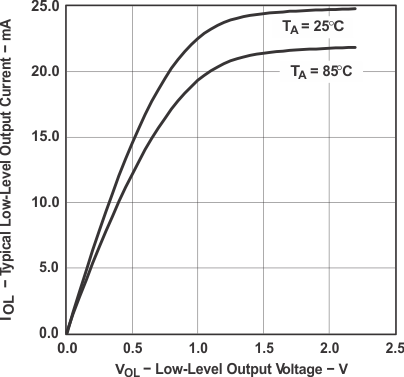Figure 4-6Figure 4-8Figure 4-7Figure 4-9

### 4.17 POR/Brownout Reset (BOR) – Electrical Characteristics(1)(2)

over recommended ranges of supply voltage and operating free-air temperature (unless otherwise noted)
PARAMETER TEST CONDITIONS MIN TYP MAX UNIT
VCC(start) See Figure 4-10 dVCC/dt ≤ 3 V/s 0.7 × V(B_IT–) V
V(B_IT–) See Figure 4-10 through Figure 4-12 dVCC/dt ≤ 3 V/s 1.71 V
Vhys(B_IT–) See Figure 4-10 dVCC/dt ≤ 3 V/s 70 130 210 mV
td(BOR) See Figure 4-10 2000 μs
t(reset) Pulse length needed at RST/NMI pin to accepted reset internally VCC = 2.2 V or 3 V 2 μs
The current consumption of the brownout module is already included in the ICC current consumption data. The voltage level V(B_IT–) + Vhys(B_IT– ) is ≤ 1.8 V.
During power up, the CPU begins code execution following a period of td(BOR) after VCC = V(B_IT–) + Vhys(B_IT–). The default DCO settings must not be changed until VCC ≥ VCC(min), where VCC(min) is the minimum supply voltage for the desired operating frequency.Figure 4-10 POR/Brownout Reset (BOR) vs Supply Voltage

### 4.18 Typical Characteristics - POR/Brownout Reset (BOR)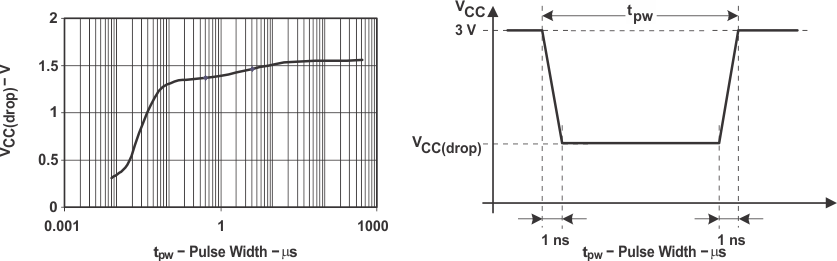Figure 4-11 VCC(drop) Level With a Square Voltage Drop to Generate a POR/Brownout Signal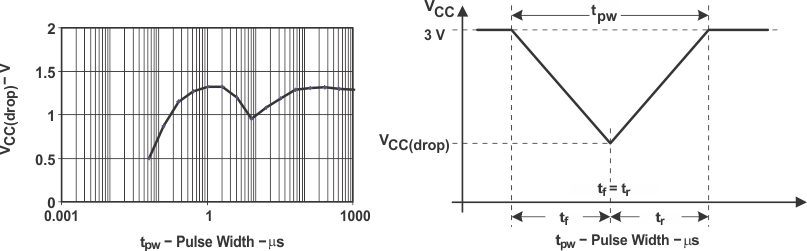Figure 4-12 VCC(drop) Level With a Triangle Voltage Drop to Generate a POR/Brownout Signal

### 4.19 SVS (Supply Voltage Supervisor/Monitor) - Electrical Characteristics

over operating free-air temperature range (unless otherwise noted)
PARAMETER TEST CONDITIONS MIN TYP MAX UNIT
t(SVSR) dVCC/dt > 30 V/ms (see Figure 4-13) 5 150 μs
dVCC/dt ≤ 30 V/ms 2000
td(SVSon) SVSON, switch from VLD = 0 to VLD ≠ 0, VCC = 3 V 20 150 μs
tsettle VLD ≠ 0 (see (1)) 12 μs
V(SVSstart) VLD ≠ 0, VCC/dt ≤ 3 V/s (see Figure 4-13) 1.55 1.7 V
Vhys(SVS_IT-) VCC/dt ≤ 3 V/s (see Figure 4-13) VLD = 1 70 120 210 mV
VLD = 2 to 14 V(SVS_IT-) × 0.004 V(SVS_IT-) × 0.016 V
VCC/dt ≤ 3 V/s (see Figure 4-13),
External voltage applied on A7
VLD = 15 4.4 20 mV
V(SVS_IT-) VCC/dt ≤ 3 V/s (see Figure 4-13 and Figure 4-14) VLD = 1 1.8 1.9 2.05 V
VLD = 2 1.94 2.1 2.25
VLD = 3 2.05 2.2 2.37
VLD = 4 2.14 2.3 2.48
VLD = 5 2.24 2.4 2.6
VLD = 6 2.33 2.5 2.71
VLD = 7 2.46 2.65 2.86
VLD = 8 2.58 2.8 3
VLD = 9 2.69 2.9 3.13
VLD = 10 2.83 3.05 3.29
VLD = 11 2.94 3.2 3.42
VLD = 12 3.11 3.35 3.61(2)
VLD = 13 3.24 3.5 3.76(2)
VLD = 14 3.43 3.7(2) 3.99(2)
VCC/dt ≤ 3 V/s (see Figure 4-13 and Figure 4-14),
External voltage applied on A7
VLD = 15 1.1 1.2 1.3
ICC(SVS)(3) VLD ≠ 0, VCC = 2.2 V/3 V 10 15 μA
tsettle is the settling time that the comparator o/p needs to have a stable level after VLD is switched VLD ≠ 0 to a different VLD value between 2 and 15. The overdrive is assumed to be > 50 mV.
The recommended operating voltage range is limited to 3.6 V.
The current consumption of the SVS module is not included in the ICC current consumption data.

### 4.20 Typical Characteristics - SVS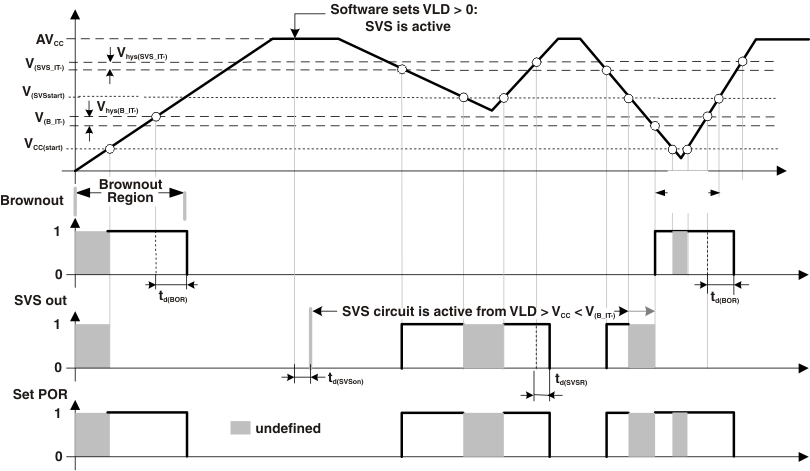Figure 4-13 SVS Reset (SVSR) vs Supply Voltage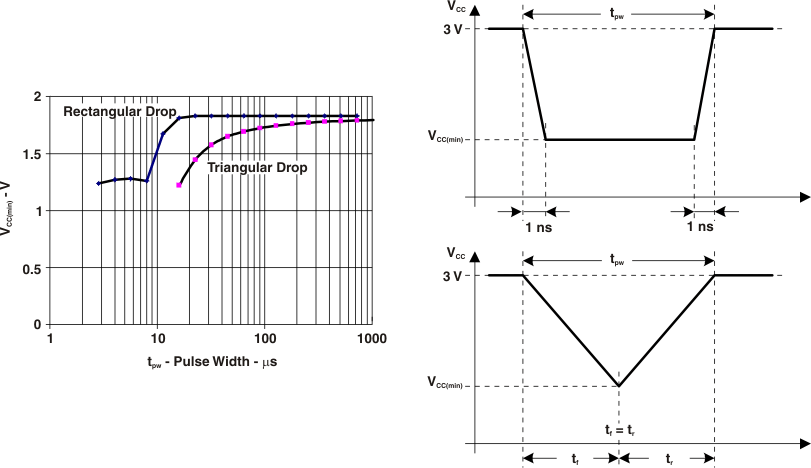Figure 4-14 VCC(min): Square Voltage Drop and Triangle Voltage Drop to Generate an SVS Signal (VLD = 1)

### 4.21 Main DCO Characteristics

• All ranges selected by RSELx overlap with RSELx + 1: RSELx = 0 overlaps RSELx = 1, ... RSELx = 14 overlaps RSELx = 15.
• DCO control bits DCOx have a step size as defined by parameter SDCO.
• Modulation control bits MODx select how often ƒDCO(RSEL,DCO+1) is used within the period of 32 DCOCLK cycles. The frequency ƒDCO(RSEL,DCO) is used for the remaining cycles. The frequency is an average equal to:
Equation 1.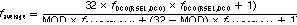### 4.22 DCO Frequency – Electrical Characteristics

over recommended ranges of supply voltage and operating free-air temperature (unless otherwise noted)
PARAMETER TEST CONDITIONS MIN TYP MAX UNIT
VCC Supply voltage range RSELx < 14 1.8 3.6 V
RSELx = 14 2.2 3.6
RSELx = 15 3.0 3.6
ƒDCO(0,0) DCO frequency (0, 0) RSELx = 0, DCOx = 0,
MODx = 0, VCC = 2.2 V or 3 V
0.06 0.14 MHz
ƒDCO(0,3) DCO frequency (0, 3) RSELx = 0, DCOx = 3,
MODx = 0, VCC = 2.2 V or 3 V
0.07 0.17 MHz
ƒDCO(1,3) DCO frequency (1, 3) RSELx = 1, DCOx = 3,
MODx = 0, VCC = 2.2 V or 3 V
0.10 0.20 MHz
ƒDCO(2,3) DCO frequency (2, 3) RSELx = 2, DCOx = 3,
MODx = 0, VCC = 2.2 V or 3 V
0.14 0.28 MHz
ƒDCO(3,3) DCO frequency (3, 3) RSELx = 3, DCOx = 3,
MODx = 0, VCC = 2.2 V or 3 V
0.20 0.40 MHz
ƒDCO(4,3) DCO frequency (4, 3) RSELx = 4, DCOx = 3,
MODx = 0, VCC = 2.2 V or 3 V
0.28 0.54 MHz
ƒDCO(5,3) DCO frequency (5, 3) RSELx = 5, DCOx = 3,
MODx = 0, VCC = 2.2 V or 3 V
0.39 0.77 MHz
ƒDCO(6,3) DCO frequency (6, 3) RSELx = 6, DCOx = 3,
MODx = 0, VCC = 2.2 V or 3 V
0.54 1.06 MHz
ƒDCO(7,3) DCO frequency (7, 3) RSELx = 7, DCOx = 3,
MODx = 0, VCC = 2.2 V or 3 V
0.80 1.50 MHz
ƒDCO(8,3) DCO frequency (8, 3) RSELx = 8, DCOx = 3,
MODx = 0, VCC = 2.2 V or 3 V
1.10 2.10 MHz
ƒDCO(9,3) DCO frequency (9, 3) RSELx = 9, DCOx = 3,
MODx = 0, VCC = 2.2 V or 3 V
1.60 3.00 MHz
ƒDCO(10,3) DCO frequency (10, 3) RSELx = 10, DCOx = 3,
MODx = 0, VCC = 2.2 V or 3 V
2.50 4.30 MHz
ƒDCO(11,3) DCO frequency (11, 3) RSELx = 11, DCOx = 3,
MODx = 0, VCC = 2.2 V or 3 V
3.00 5.50 MHz
ƒDCO(12,3) DCO frequency (12, 3) RSELx = 12, DCOx = 3,
MODx = 0, VCC = 2.2 V or 3 V
4.30 7.30 M Hz
ƒDCO(13,3) DCO frequency (13, 3) RSELx = 13, DCOx = 3,
MODx = 0, VCC = 2.2 V or 3 V
6.00 9.60 MHz
ƒDCO(14,3) DCO frequency (14, 3) RSELx = 14, DCOx = 3,
MODx = 0, VCC = 2.2 V or 3 V
8.60 13.9 MHz
ƒDCO(15,3) DCO frequency (15, 3) RSELx = 15, DCOx = 3,
MODx = 0, VCC = 2.2 V or 3 V
12.0 18.5 MHz
ƒDCO(15,7) DCO frequency (15, 7) RSELx = 15, DCOx = 7,
MODx = 0, VCC = 2.2 V or 3 V
16.0 26.0 MHz
SRSEL Frequency step between range RSEL and RSEL+1 SRSEL = ƒDCO(RSEL+1,DCO)DCO(RSEL,DCO), VCC = 2.2 V or 3 V 1.55 ratio
SDCO Frequency step between tap DCO and DCO+1 SDCO = ƒDCO(RSEL,DCO+1)DCO(RSEL,DCO), VCC = 2.2 V or 3 V 1.05 1.08 1.12 ratio
Duty cycle Measured at P1.4/SMCLK, VCC = 2.2 V or 3 V 40% 50% 60%

### 4.23 Calibrated DCO Frequencies (Tolerance at Calibration) – Electrical Characteristics

over recommended ranges of supply voltage and operating free-air temperature (unless otherwise noted)
PARAMETER TEST CONDITIONS MIN TYP MAX UNIT
Frequency tolerance at calibration TA = 25°C, VCC = 3 V –1% ±0.2% 1%
ƒCAL(1 MHz) 1-MHz calibration value BCSCTL1 = CALBC1_1MHZ,
DCOCTL = CALDCO_1MHZ,
Gating time: 5 ms
TA = 25°C, VCC = 3 V
0.990 1 1.010 MHz
ƒCAL(8 MHz) 8-MHz calibration value BCSCTL1 = CALBC1_8MHZ,
DCOCTL = CALDCO_8MHZ,
Gating time: 5 ms
TA = 25°C, VCC = 3 V
7.920 8 8.080 MHz
ƒCAL(12 MHz) 12-MHz calibration value BCSCTL1 = CALBC1_12MHZ,
DCOCTL = CALDCO_12MHZ,
Gating time: 5 ms
TA = 25°C, VCC = 3 V
11.88 12 12.12 MHz
ƒCAL(16 MHz) 16-MHz calibration value BCSCTL1 = CALBC1_16MHZ,
DCOCTL = CALDCO_16MHZ,
Gating time: 2 ms
TA = 25°C, VCC = 3 V
15.84 16 16.16 MHz

### 4.24 Calibrated DCO Frequencies (Tolerance Over Temperature) – Electrical Characteristics

over recommended ranges of supply voltage and operating free-air temperature (unless otherwise noted)
PARAMETER TEST CONDITIONS MIN TYP MAX UNIT
1-MHz tolerance over temperature TA = 0°C to 85°C, VCC = 3 V –2.5% ±0.5% 2.5%
8-MHz tolerance over temperature TA = 0°C to 85°C, VCC = 3 V –2.5% ±1.0% 2.5%
12-MHz tolerance over temperature TA = 0°C to 85°C, VCC = 3 V –2.5% ±1.0% 2.5%
16-MHz tolerance over temperature TA = 0°C to 85°C, VCC = 3 V –3.0% ±2.0% 3.0%
ƒCAL(1MHz) 1-MHz calibration value BCSCTL1 = CALBC1_1MHz,
DCOCTL = CALDCO_1MHZ,
Gating time: 5 ms
TA = 0°C to 85°C
VCC = 2.2 V 0.970 1 1.030 MHz
VCC = 3 V 0.975 1 1.025
VCC = 3.6 V 0.970 1 1.030
ƒCAL(8MHz) 8-MHz calibration value BCSCTL1 = CALBC1_8MHZ,
DCOCTL = CALDCO_8MHZ,
Gating time: 5 ms
TA = 0°C to 85°C
VCC = 2.2 V 7.760 8 8.400 MHz
VCC = 3 V 7.800 8 8.200
VCC = 3.6 V 7.600 8 8.240
ƒCAL(12MHz) 12-MHz calibration value BCSCTL1 = CALBC1_12MHZ,
DCOCTL = CALDCO_12MHZ,
Gating time: 5 ms
TA = 0°C to 85°C
VCC = 2.2 V 11.70 12 12.30 MHz
VCC = 3 V 11.70 12 12.30
VCC = 3.6 V 11.70 12 12.30
ƒCAL(16MHz) 16-MHz calibration value BCSCTL1 = CALBC1_16MHZ,
DCOCTL = CALDCO_16MHZ,
Gating time: 2 ms
TA = 0°C to 85°C
VCC = 3 V 15.52 16 16.48 MHz
VCC = 3.6 V 15.00 16 16.48

### 4.25 Calibrated DCO Frequencies (Tolerance Over Supply Voltage VCC) – Electrical Characteristics

over recommended ranges of supply voltage and operating free-air temperature (unless otherwise noted)
PARAMETER TEST CONDITIONS MIN TYP MAX UNIT
1-MHz tolerance over VCC TA = 25°C, VCC = 1.8 V to 3.6 V –3% ±2% 3%
8-MHz tolerance overVCC TA = 25°C, VCC = 1.8 V to 3.6 V –3% ±2% 3%
12-MHz tolerance over VCC TA = 25°C, VCC = 2.2 V to 3.6 V –3% ±2% 3%
16-MHz tolerance over VCC TA = 25°C, VCC = 3 V to 3.6 V –6% ±2% 3%
ƒCAL(1MHz) 1-MHz
calibration value
BCSCTL1 = CALBC1_1MHZ, DCOCTL = CALDCO_1MHZ,
Gating time: 5 ms, TA = 25°C, VCC = 1.8 V to 3.6 V
0.970 1 1.030 MHz
ƒCAL(8MHz) 8-MHz
calibration value
BCSCTL1 = CALBC1_8MHZ, DCOCTL = CALDCO_8MHZ,
Gating time: 5 ms, TA = 25°C, VCC = 1.8 V to 3.6 V
7.760 8 8.240 MHz
ƒCAL(12MHz) 12-MHz
calibration value
BCSCTL1 = CALBC1_12MHZ, DCOCTL = CALDCO_12MHZ,
Gating time: 5 ms, TA = 25°C, VCC = 2.2 V to 3.6 V
11.64 12 12.36 MHz
ƒCAL(16MHz) 16-MHz
calibration value
BCSCTL1 = CALBC1_16MHZ, DCOCTL = CALDCO_16MHZ,
Gating time: 2 ms, TA = 25°C, VCC = 3 V to 3.6 V
15.00 16 16.48 MHz

### 4.26 Calibrated DCO Frequencies (Overall Tolerance) – Electrical Characteristics

over recommended ranges of supply voltage and operating free-air temperature (unless otherwise noted)
PARAMETER TEST CONDITIONS MIN TYP MAX UNIT
1-MHz tolerance
over temperature
TA = –55°C to 150°C, VCC = 1.8 V to 3.6 V –5% ±2% 5%
8-MHz tolerance
over temperature
TA = –55°C to 150°C, VCC = 1.8 V to 3.6 V –5% ±2% 5%
12-MHz tolerance
over temperature
TA = –55°C to 150°C, VCC = 2.2 V to 3.6 V –5% ±2% 5%
16-MHz tolerance
over temperature
TA = –55°C to 150°C, VCC = 3 V to 3.6 V –6% ±3% 6%
ƒCAL(1MHz) 1-MHz
calibration value
BCSCTL1 = CALBC1_1MHZ, DCOCTL = CALDCO_1MHZ,
Gating time: 5 ms, TA = –55°C to 150°C, VCC = 1.8 V to 3.6 V
.950 1 1.050 MHz
ƒCAL(8MHz) 8-MHz
calibration value
BCSCTL1 = CALBC1_8MHZ, DCOCTL = CALDCO_8MHZ,
Gating time: 5 ms, TA = –55°C to 150°C, VCC = 1.8 V to 3.6 V
7.6 8 8.4 MHz
ƒCAL(12MHz) 12-MHz
calibration value
BCSCTL1 = CALBC1_12MHZ, DCOCTL = CALDCO_12MHZ,
Gating time: 5 ms, TA = –55°C to 150°C, VCC = 2.2 V to 3.6 V
11.4 12 12.6 MHz
ƒCAL(16MHz) 16-MHz
calibration value
BCSCTL1 = CALBC1_16MHZ, DCOCTL = CALDCO_16MHZ,
Gating time: 2 ms, TA = –55°C to 150°C, VCC = 3 V to 3.6 V
15.00 16 17.00 MHz

### 4.27 Typical Characteristics – Calibrated DCO Frequency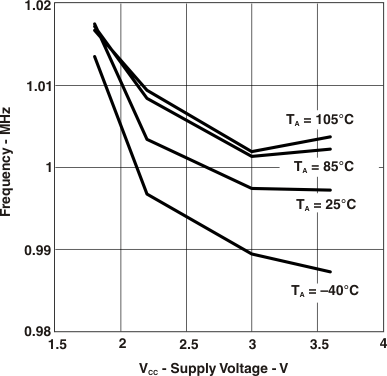Figure 4-15 Calibrated 1-MHz DCO Frequency vs VCC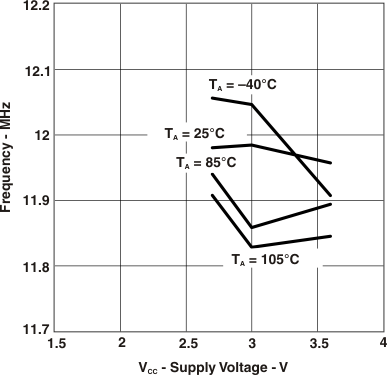Figure 4-17 Calibrated 12-MHz DCO Frequency vs VCCFigure 4-16 Calibrated 8-MHz DCO Frequency vs VCC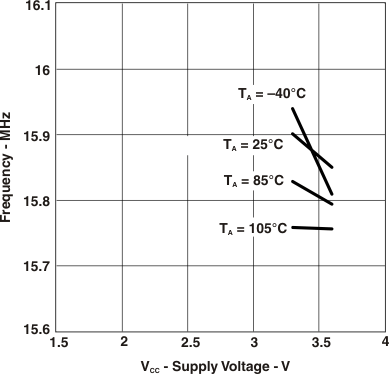Figure 4-18 Calibrated 16-MHz DCO Frequency vs VCC

### 4.28 Wake-Up From Low-Power Modes (LPM3/4) – Electrical Characteristics

over recommended ranges of supply voltage and operating free-air temperature (unless otherwise noted)
PARAMETER TEST CONDITIONS MIN TYP MAX UNIT
tDCO,LPM3/4 DCO clock wake-up time
from LPM3/4(1)
BCSCTL1 = CALBC1_1MHZ,
DCOCTL = CALDCO_1MHZ,
VCC = 2.2 V or 3 V
2 μs
BCSCTL1 = CALBC1_8MHZ,
DCOCTL = CALDCO_8MHZ,
VCC = 2.2 V or 3 V
1.5
BCSCTL1 = CALBC1_12MHZ,
DCOCTL = CALDCO_12MHZ,
VCC = 3 V
1
BCSCTL1 = CALBC1_16MHZ,
DCOCTL = CALDCO_16MHZ,
VCC = 3 V
1
tCPU,LPM3/4 CPU wake-up time from LPM3/4(2) 1/ƒMCLK +
tClock,LPM3/4
The DCO clock wake-up time is measured from the edge of an external wake-up signal (for example, port interrupt) to the first clock edge observable externally on a clock pin (MCLK or SMCLK).
Parameter applicable only if DCOCLK is used for MCLK.

### 4.29 Typical Characteristics – DCO Clock Wake-Up Time From LPM3/4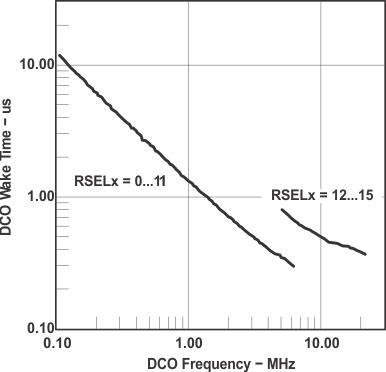Figure 4-19 Clock Wake-Up Time From LPM3 vs DCO Frequency

### 4.30 DCO With External Resistor ROSC – Electrical Characteristics(1)

over recommended ranges of supply voltage and operating free-air temperature (unless otherwise noted)
PARAMETER TEST CONDITIONS TYP UNIT
ƒDCO,ROSC DCO output frequency with ROSC DCOR = 1, RSELx = 4, DCOx = 3,
MODx = 0, TA = 25°C
VCC = 2.2 V 1.8 MHz
VCC = 3 V 1.95
Dt Temperature drift DCOR = 1, RSELx = 4, DCOx = 3, MODx = 0,
VCC = 2.2 V or 3 V
±0.1 %/°C
DV Drift with VCC DCOR = 1, RSELx = 4, DCOx = 3, MODx = 0,
VCC = 2.2 V or 3 V
10 %/V
ROSC = 100 kΩ. Metal film resistor, type 0257. 0.6 watt with 1% tolerance and TK = ±50 ppm/°C

### 4.31 Typical Characteristics - DCO With External Resistor ROSC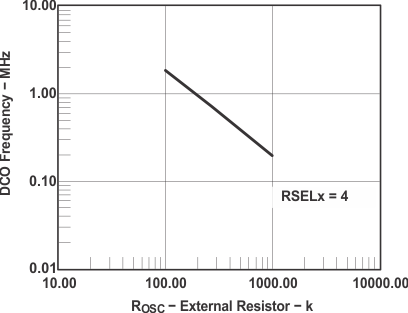Figure 4-20 DCO Frequency vs ROSC,
VCC = 2.2 V, TA = 25°C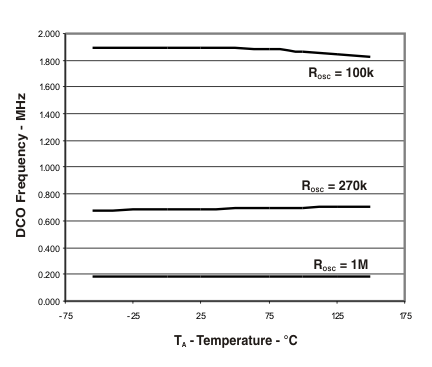Figure 4-22 DCO Frequency vs Temperature,
VCC = 3.0 V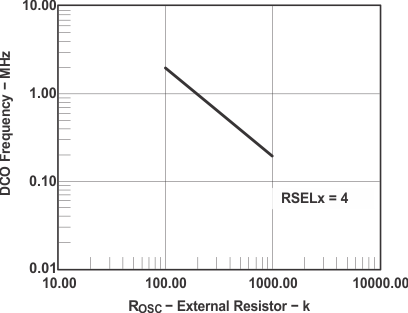Figure 4-21 DCO Frequency vs ROSC,
VCC = 3.0 V, TA = 25°C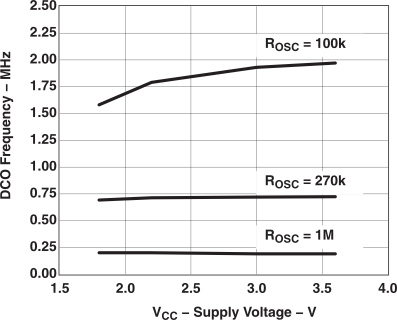Figure 4-23 DCO Frequency vs VCC,
TA = 25°C

### 4.32 Crystal Oscillator (LFXT1) Low-Frequency Modes – Electrical Characteristics(1)(2)

over recommended ranges of supply voltage and operating free-air temperature (unless otherwise noted)
PARAMETER TEST CONDITIONS MIN TYP MAX UNIT
ƒLFXT1,LF LFXT1 oscillator crystal frequency, LF mode 0, 1 XTS = 0, LFXT1Sx = 0 or 1, VCC = 1.8 V to 3.6 V 32,768 Hz
ƒLFXT1,LF,logic LFXT1 oscillator logic-level square-wave input frequency, LF mode XTS = 0, LFXT1Sx = 3, VCC = 1.8 V to 3.6 V 10,000 32,768 50,000 Hz
OALF Oscillation allowance for LF crystals XTS = 0, LFXT1Sx = 0; ƒLFXT1,LF = 32,768 kHz,
CL,eff = 6 pF
500
XTS = 0, LFXT1Sx = 0;ƒLFXT1,LF = 32,768 kHz,
CL,eff = 12 pF
200
LF mode(3)
XTS = 0 XCAPx = 0 1 pF
XCAPx = 1 5.5
XCAPx = 2 8.5
XCAPx = 3 11
Duty cycle LF mode XTS = 0, Measured at P1.4/ACLK,
ƒLFXT1,LF = 32,768 Hz, VCC = 2.2 V or 3 V
30% 50% 70%
ƒFault,LF Oscillator fault frequency threshold, LF mode (5) XTS = 0, LFXT1Sx = 3(4), VCC = 2.2 V or 3 V 10 10,000 Hz
To improve EMI on the LFXT1 oscillator the following guidelines should be observed:
• Keep as short of a trace as possible between the device and the crystal.
• Design a good ground plane around the oscillator pins.
• Prevent crosstalk from other clock or data lines into oscillator pins XIN and XOUT.
• Avoid running PCB traces underneath or adjacent to the XIN and XOUT pins.
• Use assembly materials and praxis to avoid any parasitic load on the oscillator XIN and XOUT pins.
• If conformal coating is used, ensure that it does not induce capacitive/resistive leakage between the oscillator pins.
• Do not route the XOUT line to the JTAG header to support the serial programming adapter as shown in other documentation. This signal is no longer required for the serial programming adapter.
LFXT1 in 32-KHz mode is specified to function only between –55°C to 105°C. This module is know to fail above 110°C. For further info contact TI support.
Includes parasitic bond and package capacitance (approximately 2 pF per pin). Since the PCB adds additional capacitance it is recommended to verify the correct load by measuring the ACLK frequency. For a correct setup the effective load capacitance should always match the specification of the used crystal.
Measured with logic-level input frequency, but also applies to operation with crystals.
Frequencies below the MIN specification set the fault flag, frequencies above the MAX specification do not set the fault flag. Frequencies in between might set the flag.

### 4.33 Internal Very-Low-Power, Low-Frequency Oscillator (VLO) – Electrical Characteristics

over recommended ranges of supply voltage and operating free-air temperature (unless otherwise noted)
PARAMETER TEST CONDITIONS MIN TYP MAX UNIT
ƒVLO VLO frequency TA = –55°C to 85°C, VCC = 2.2 V or 3 V 4 12 20 kHz
TA = 150°C, VCC = 2.2 V or 3 V 22
VLO/dT VLO frequency temperature drift See (1), VCC = 2.2 V or 3 V 0.5 0.8 %/°C
VLO/dVCC VLO frequency supply voltage drift See (2), TA = 25°C, VCC = 1.8 V to 3.6V 4 %/V
Calculated using the box method:
S Version: (MAX(–55 to 150°C) – MIN(–55 to 150°C))/MIN(–55 to 150°C)/(150°C – (–55°C))
Calculated using the box method: (MAX(1.8 V to 3.6 V) – MIN(1.8V to 3.6 V))/MIN(1.8 V to 3.6 V)/(3.6 V – 1.8 V)

### 4.34 Crystal Oscillator (LFXT1) High Frequency Modes – Electrical Characteristics(5)

over recommended ranges of supply voltage and operating free-air temperature (unless otherwise noted)
PARAMETER TEST CONDITIONS MIN TYP MAX UNIT
ƒLFXT1,HF0 LFXT1 oscillator crystal frequency,
HF mode 0
XTS = 1, LFXT1Sx = 0, VCC = 1.8 V to 3.6 V 0.4 1 MHz
ƒLFXT1,HF1 LFXT1 oscillator lcrystal frequency,
HF mode 1
XTS = 1, LFXT1Sx = 1, VCC = 1.8 V to 3.6 V 1 4 MHz
ƒLFXT1,HF2 LFXT1 oscillator crystal frequency,
HF mode 2
XTS = 1, LFXT1Sx = 2 VCC = 1.8 V to 3.6 V 2 10 MHz
VCC = 2.2 V to 3.6 V 2 12
VCC = 3 V to 3.6 V 2 16
ƒLFXT1,HF,logic LFXT1 oscillator logic-level
square-wave input frequency,
HF mode
XTS = 1, LFXT1Sx = 3 VCC = 1.8 V to 3.6 V 0.4 10 MHz
VCC = 2.2 V to 3.6 V 0.4 12
VCC = 3 V to 3.6 V 0.4 16
OAHF Oscillation allowance for HF crystals
(see Figure 4-24 and Figure 4-25)
XTS = 0, LFXT1Sx = 0;
ƒLFXT1,HF = 1 MHz, CL,eff = 15 pF
2700 Ω
XTS = 0, LFXT1Sx = 1
ƒLFXT1,HF = 4 MHz, CL,eff = 15 pF
800
XTS = 0, LFXT1Sx = 2
ƒLFXT1,HF = 16 MHz, CL,eff = 15 pF
300
HF mode(1)
XTS = 1(2) 1 pF
Duty cycle HF mode XTS = 1, Measured at P1.4/ACLK,
ƒLFXT1,HF = 10 MHz, VCC = 3 V
40% 50% 60%
XTS = 1, Measured at P1.4/ACLK,
ƒLFXT1,HF = 16 MHz, VCC = 3 V
40% 50% 60%
ƒFault,HF Oscillator fault frequency, HF mode(4) XTS = 1, LFXT1Sx = 3(3), VCC = 2.2 V or 3 V 30 300 kHz
Includes parasitic bond and package capacitance (approximately 2 pF per pin). Since the PCB adds additional capacitance it is recommended to verify the correct load by measuring the ACLK frequency. For a correct setup the effective load capacitance should always match the specification of the used crystal.
Requires external capacitors at both terminals. Values are specified by crystal manufacturers.
Measured with logic-level input frequency, but also applies to operation with crystals
Frequencies below the MIN specification set the fault flag, frequencies above the MAX specification do not set the fault flag. Frequencies in between might set the flag.
To improve EMI on the LFXT1 oscillator the following guidelines should be observed:
• Keep as short of a trace as possible between the device and the crystal.
• Design a good ground plane around the oscillator pins.
• Prevent crosstalk from other clock or data lines into oscillator pins XIN and XOUT.
• Avoid running PCB traces underneath or adjacent to the XIN and XOUT pins.
• Use assembly materials and praxis to avoid any parasitic load on the oscillator XIN and XOUT pins.
• If conformal coating is used, ensure that it does not induce capacitive/resistive leakage between the oscillator pins.
• Do not route the XOUT line to the JTAG header to support the serial programming adapter as shown in other documentation. This signal is no longer required for the serial programming adapter.

### 4.35 Typical Characteristics – LFXT1 Oscillator in HF Mode (XTS = 1)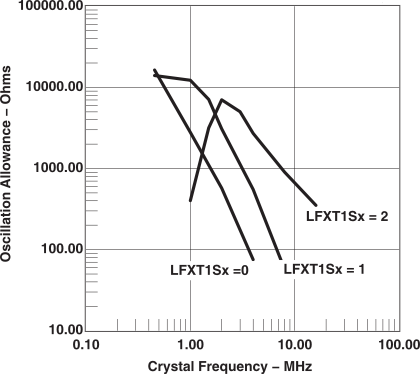Figure 4-24 Oscillation Allowance vs Crystal Frequency, CL,eff = 15 pF, TA = 25°C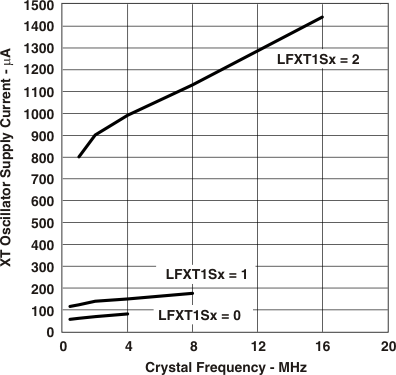Figure 4-25 XT Oscillator Supply Current vs Crystal Frequency, CL,eff = 15 pF, TA = 25°C

### 4.36 Crystal Oscillator (XT2) – Electrical Characteristics(5)

over recommended ranges of supply voltage and operating free-air temperature (unless otherwise noted)
PARAMETER TEST CONDITIONS MIN TYP MAX UNIT
ƒXT2 XT2 oscillator crystal frequency,
mode 0
XT2Sx = 0, VCC = 1.8 V to 3.6 V 0.4 0.9 MHz
ƒXT2 XT2 oscillator lcrystal frequency,
mode 1
XT2Sx = 1, VCC = 1.8 V to 3.6 V 1 4 MHz
ƒXT2 XT2 oscillator crystal frequency,
mode 2
XT2Sx = 2 VCC = 1.8 V to 3.6 V 2 10 MHz
VCC = 2.2 V to 3.6 V 2 12
VCC = 3 V to 3.6 V 2 16
ƒXT2 XT2 oscillator logic-level
square-wave input frequency,
XT2Sx = 3 VCC = 1.8 V to 3.6 V 0.4 10 MHz
VCC = 2.2 V to 3.6 V 0.4 12
VCC = 3 V to 3.6 V 0.4 16
OA Oscillation allowance
(see Figure 4-26 and Figure 4-27)
XT2Sx = 0, ƒXT2 = 1 MHz; CL,eff = 15 pF 2700 Ω
XT2Sx = 1, ƒXT2 = 4MHz; CL,eff = 15 pF 800
XT2Sx = 2, ƒXT1, HF = 16 MHz; CL,eff = 15 pF 300
HF mode(1)
See (2) 1 pF
Duty cycle HF mode Measured at P1.4/SMCLK,
ƒXT2 = 10 MHz, VCC = 2.2 V or 3 V
40% 50% 60%
Measured at P1.4/SMCLK,
ƒXT2 = 16 MHz, VCC = 2.2 V or 3 V
40% 50% 60%
ƒFault Oscillator fault frequency, HF mode(4) XT2Sx = 3(3), VCC = 2.2 V or 3 V 30 300 kHz
Includes parasitic bond and package capacitance (approximately 2 pF per pin). Since the PCB adds additional capacitance it is recommended to verify the correct load by measuring the ACLK frequency. For a correct setup the effective load capacitance should always match the specification of the used crystal.
Requires external capacitors at both terminals. Values are specified by crystal manufacturers.
Measured with logic-level input frequency, but also applies to operation with crystals.
Frequencies below the MIN specification set the fault flag, frequencies above the MAX specification do not set the fault flag. Frequencies in between might set the flag.
To improve EMI on the LFXT1 oscillator the following guidelines should be observed:
• Keep as short of a trace as possible between the device and the crystal.
• Design a good ground plane around the oscillator pins.
• Prevent crosstalk from other clock or data lines into oscillator pins XIN and XOUT.
• Avoid running PCB traces underneath or adjacent to the XIN and XOUT pins.
• Use assembly materials and praxis to avoid any parasitic load on the oscillator XIN and XOUT pins.
• If conformal coating is used, ensure that it does not induce capacitive/resistive leakage between the oscillator pins.
• Do not route the XOUT line to the JTAG header to support the serial programming adapter as shown in other documentation. This signal is no longer required for the serial programming adapter.

### 4.37 Typical Characteristics – XT2 Oscillator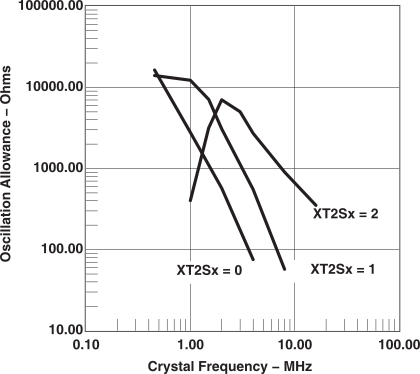Figure 4-26 Oscillation Allowance vs Crystal Frequency, CL,eff = 15 pF, TA = 25°C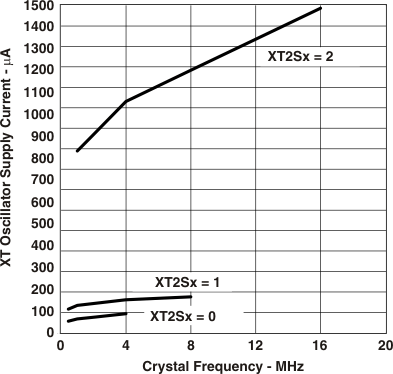Figure 4-27 XT2 Oscillator Supply Current vs Crystal Frequency, CL,eff = 15 pF, TA = 25°C

### 4.38 Timer_A – Electrical Characteristics

over recommended ranges of supply voltage and operating free-air temperature (unless otherwise noted)
PARAMETER TEST CONDITIONS MIN MAX UNIT
ƒTA Timer_A clock frequency Internal: SMCLK, ACLK,
External: TACLK, INCLK,
Duty cycle = 50% ±10%
VCC = 2.2 V 10 MHz
VCC = 3 V 16
tTA,cap Timer_A, capture timing TA0, TA1, TA2, VCC = 2.2 V or 3 V 20 ns

### 4.39 Timer_B – Electrical Characteristics

over recommended ranges of supply voltage and operating free-air temperature (unless otherwise noted)
PARAMETER TEST CONDITIONS MIN MAX UNIT
ƒTB Timer_B clock frequency Internal: SMCLK, ACLK,
External: TBCLK,
Duty cycle = 50% ±10%
VCC = 2.2 V 10 MHz
VCC = 3 V 16
tTB,cap Timer_B, capture timing TB0, TB1, TB2, VCC = 2.2 V or 3 V 20 ns

### 4.40 USCI (UART Mode) – Electrical Characteristics

over recommended ranges of supply voltage and operating free-air temperature (unless otherwise noted)
PARAMETER TEST CONDITIONS MIN TYP MAX UNIT
ƒUSCI USCI input clock frequency Internal: SMCLK, ACLK,
External: UCLK;
Duty cycle = 50% ±10%
ƒSYSTEM MHz
ƒBITCLK BITCLK clock frequency
(equals baud rate in MBaud)
VCC = 2.2 V or 3 V 1 MHz
tτ UART receive deglitch time(1) VCC = 2.2 V 50 150 600 ns
VCC = 3 V 50 150 600
Pulses on the UART receive input (UCxRX) shorter than the UART receive deglitch time are suppressed. To ensure that pulses are correctly recognized, their width should exceed the maximum specification of the deglitch time.

### 4.41 USCI (SPI Master Mode) – Electrical Characteristics

over recommended ranges of supply voltage and operating free-air temperature (unless otherwise noted)(1) (see Figure 4-28 and Figure 4-29)
PARAMETER TEST CONDITIONS MIN MAX UNIT
ƒUSCI USCI input clock frequency SMCLK, ACLK, Duty cycle = 50% ±10% ƒSYSTEM MHz
tSU,MI SOMI input data setup time VCC= 2.2 V 110 ns
VCC = 3 V 75
tHD,MI SOMI input data hold time VCC = 2.2 V 0 ns
VCC = 3 V 0
tVALID,MO SIMO output data valid time UCLK edge to SIMO valid,
CL = 20 pF
VCC = 2.2 V 30 ns
VCC = 3 V 20
ƒUCxCLK = 1/2tLO/HI with tLO/HI ≥ max(tVALID,MO(USCI) + tSU,SI(Slave), tSU,MI(USCI) + tVALID,SO(Slave)).
For the slave parameters tSU,SI(Slave) and tVALID,SO(Slave), see the SPI parameters of the attached slave.

### 4.42 USCI (SPI Slave Mode) – Electrical Characteristics

over recommended ranges of supply voltage and operating free-air temperature (unless otherwise noted)(1) (see Figure 4-30 and Figure 4-31)
PARAMETER TEST CONDITIONS MIN TYP MAX UNIT
STE low to clock
VCC = 2.2 V or 3 V 50 ns
tSTE,LAG STE lag time,
Last clock to STE high
VCC = 2.2 V or 3 V 10 ns
tSTE,ACC STE access time,
STE low to SOMI data out
VCC = 2.2 V or 3 V 50 ns
tSTE,DIS STE disable time,
STE high to SOMI high impedance
VCC = 2.2 V or 3 V 50 ns
tSU,SI SIMO input data setup time VCC = 2.2 V 20 ns
VCC = 3 V 15
tHD,SI SIMO input data hold time VCC = 2.2 V 10 ns
VCC = 3 V 10
tVALID,SO SOMI output data valid time UCLK edge to SOMI valid,
CL = 20 pF
VCC = 2.2 V 75 110 ns
VCC = 3 V 50 75
ƒUCxCLK = 1/2tLO/HI with tLO/HI ≥ max(tVALID,MO(USCI) + tSU,SI(Slave), tSU,MI(USCI) + tVALID,SO(Slave)).
For the slave parameters tSU,SI(Slave) and tVALID,SO(Slave), see the SPI parameters of the attached slave.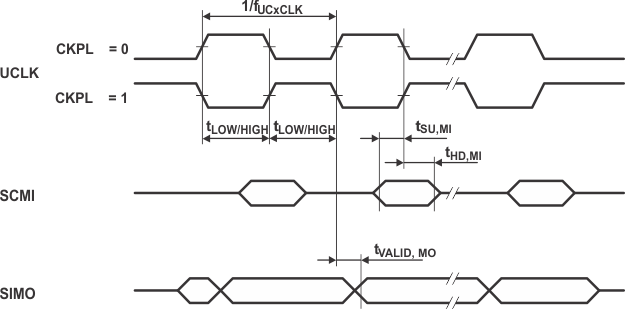Figure 4-28 SPI Master Mode, CKPH = 0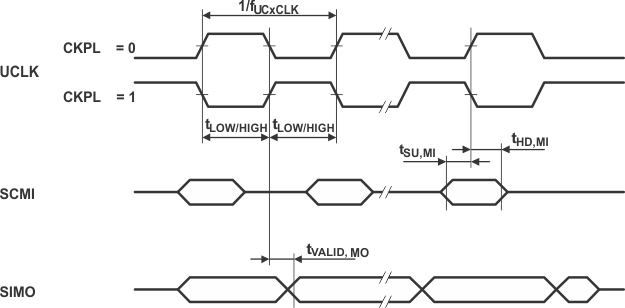Figure 4-29 SPI Master Mode, CKPH = 1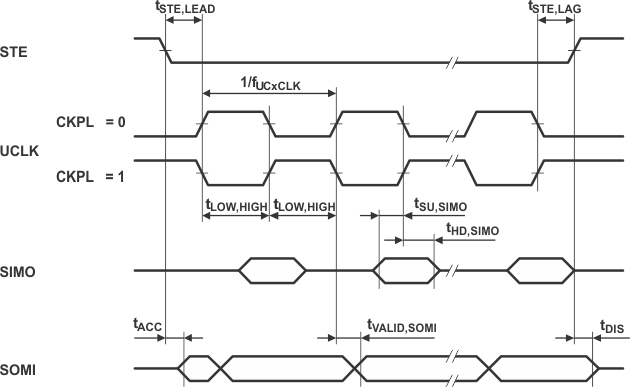Figure 4-30 SPI Slave Mode, CKPH = 0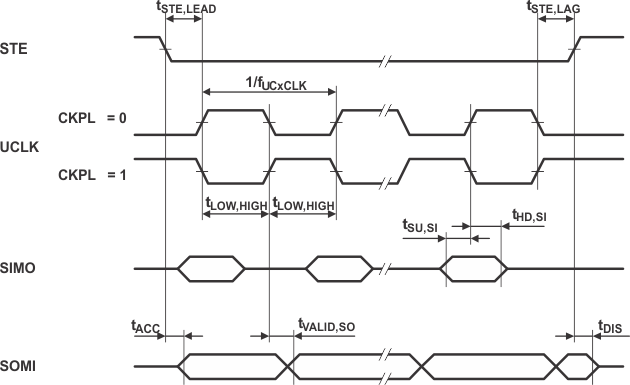Figure 4-31 SPI Slave Mode, CKPH = 1

### 4.43 USCI (I2C Mode) – Electrical Characteristics

over recommended ranges of supply voltage and operating free-air temperature (unless otherwise noted) (see Figure 4-32)
PARAMETER TEST CONDITIONS MIN TYP MAX UNIT
ƒUSCI USCI input clock frequency Internal: SMCLK, ACLK,
External: UCLK,
Duty cycle = 50% ± 10%
ƒSYSTEM MHz
ƒSCL SCL clock frequency VCC = 2.2 V or 3 V 0 400 kHz
tHD,STA Hold time (repeated) START ƒSCL ≤ 100 kHz, VCC = 2.2 V or 3 V 4.0 μs
ƒSCL > 100 kHz, VCC = 2.2 V or 3 V 0.6
tSU,STA Set-up time for a repeated START ƒSCL ≤ 100 kHz, VCC = 2.2 V or 3 V 4.7 μs
ƒSCL > 100 kHz, VCC = 2.2 V or 3 V 0.6
tHD,DAT Data hold time VCC = 2.2 V or 3 V 0 ns
tSU,DAT Data set-up time VCC = 2.2 V or 3 V 250 ns
tSU,STO Set-up time for STOP VCC = 2.2 V or 3 V 4.0 μs
tSP Pulse width of spikes suppressed by input filter VCC = 2.2 V 50 150 600 ns
VCC = 3 V 50 100 600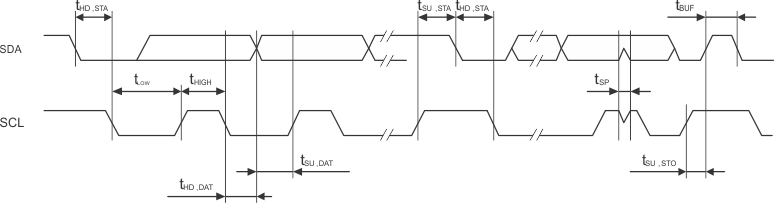Figure 4-32 I2C Mode Timing

### 4.44 Comparator_A+ – Electrical Characteristics(1)

over recommended ranges of supply voltage and operating free-air temperature (unless otherwise noted)
PARAMETER TEST CONDITIONS MIN TYP MAX UNIT
I(DD) CAON = 1 CARSEL = 0 CAREF = 0 VCC = 2.2 V 25 80 μA
VCC = 3 V 45 96
I(Refladder/Refdiode) CAON = 1, CARSEL = 0,
CAREF = 1/2/3 no load at P2 3/CA0/TA1
and P2.4/CA1/TA2
VCC = 2.2 V or 3 V 30 50 μA
VCC = 3 V 45 71
V(IC) Common-mode input voltage CAON =1, VCC = 2.2 V or 3 V 0 VCC - 1 V
V(Ref025) Voltage at 0.25 VCC node/VCC PCA0 = 1, CARSEL = 1, CAREF = 1,
and P2.4/CA1/TA2, VCC = 2.2 V or 3 V
0.23 0.24 0.25 V
V(Ref050) Voltage at 0.5 VCC node/VCC PCA0 = 1, CARSEL = 1, CAREF = 2,
and P2.4/CA1/TA2, VCC = 2.2 V or 3 V
0.47 0.48 0.5 V
V(RefVT) See Figure 4-36 and Figure 4-37 PCA0 = 1, CARSEL = 1,
CAREF = 3, no load at P2.3/CA0/TA1
and P2.4/CA1/TA2, TA = 85°C
VCC = 2.2 V 390 480 540 mV
VCC = 3 V 400 490 550
V(offset) Offset voltage See (2), VCC = 2.2 V or 3 V –30 30 mV
Vhys Input hysteresis CAON=1, VCC = 2.2 V or 3 V 0 0.7 1.4 mV
t(response) Response time, low-to-high and high-to-low(3) TA = 25°C, Overdrive 10 mV,
Without filter: CAF = 0
VCC = 2.2 V 80 165 300 ns
VCC = 3 V 70 120 240
TA = 25°C, Overdrive 10 mV,
Without filter: CAF = 1
VCC = 2.2 V 1.4 1.9 2.8 µs
VCC = 3 V 0.9 1.5 2.2
The leakage current for the Comparator_A+ terminals is identical to Ilkg(Px.x) specification.
The input offset voltage can be cancelled by using the CAEX bit to invert the Comparator_A+ inputs on successive measurements.
The two successive measurements are then summed together.
The response time is measured at P2.2/CAOUT/TA0/CA4 with an input voltage step, with Comparator_A+ already enabled (CAON = 1). If CAON is set at the same time, a settling time of up to 300 ns is added to the response time.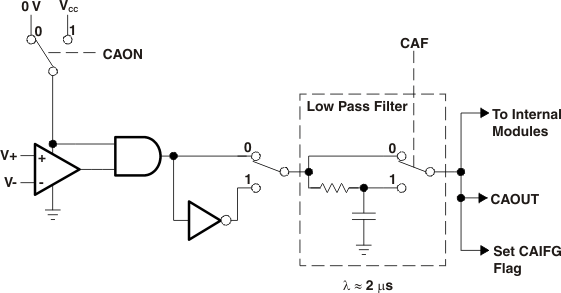Figure 4-33 Block Diagram of Comparator_A Module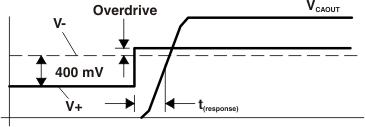Figure 4-34 Overdrive Definition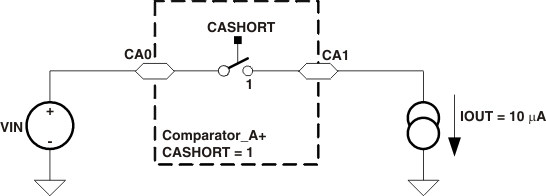Figure 4-35 Comparator_A+ Short Resistance Test Condition

### 4.45 Typical Characteristics – Comparator A+Figure 4-36 V(RefVT) vs Temperature, VCC = 3 V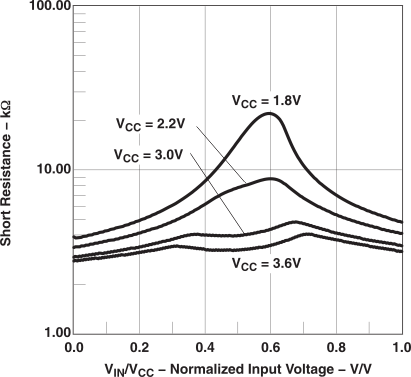Figure 4-38 Short Resistance vs VIN/VCC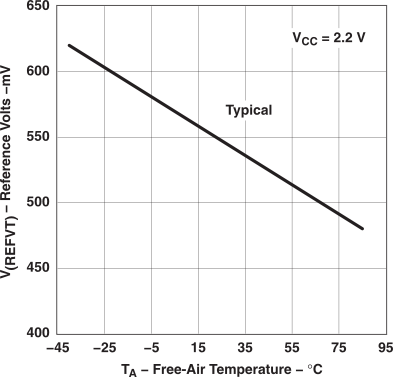Figure 4-37 V(RefVT) vs Temperature, VCC = 2.2 V

### 4.46 12-Bit ADC Power-Supply and Input Range Conditions – Electrical Characteristics(1)

over recommended ranges of supply voltage and operating free-air temperature (unless otherwise noted)
PARAMETER TEST CONDITIONS MIN TYP MAX UNIT
AVCC Analog supply voltage range AVCC and DVCC are connected together,
AVSS and DVSS are connected together,
V(AVSS) = V(DVSS) = 0 V
2.2 3.6 V
and P6Sel.x = 1, 0 ≤ x ≤ 7,
V(AVSS) ≤ VP6.x/Ax ≤ V(AVCC)
0 VAVCC V
into AVCC terminal(3)
ADC12ON = 1, REFON = 0,
SHT0 = 0, SHT1 = 0,
VCC = 2.2 V 0.65 0.8 mA
VCC = 3 V 0.8 1
IREF+ Reference supply current,
into AVCC terminal(4)
REFON = 1, REF2_5V = 1, VCC = 3 V
0.5 0.7 mA
REFON = 1, REF2_5V = 0
VCC = 2.2 V 0.5 0.7
VCC = 3 V 0.5 0.7
CI(5) Input capacitance Only one terminal selected at a time, P6.x/Ax, VCC = 2.2 V 40 pF
RI(5) Input MUX ON resistance 0 V ≤ VAx ≤ VAVCC, VCC = 3 V 2000 Ω
The leakage current is defined in the leakage current table with P6.x/Ax parameter.
The analog input voltage range must be within the selected reference voltage range VR+ to VR– for valid conversion results.
The internal reference supply current is not included in current consumption parameter IADC12.
The internal reference current is supplied via terminal VCC. Consumption is independent of the ADC10ON control bit, unless a conversion is active. The REFON bit enables the built-in reference to settle before starting an A/D conversion.
Limits verified by design.

### 4.47 12-Bit ADC External Reference – Electrical Characteristics

over recommended ranges of supply voltage and operating free-air temperature (unless otherwise noted)
PARAMETER TEST CONDITIONS MIN TYP MAX UNIT
VeREF+ Positive external reference voltage input VeREF+ > VREF-/VeREF- (1) 1.4 VAVCC V
VREF- /VeREF+ Negative external reference voltage input VeREF+ > VREF-/VeREF- (2) 0 1.2 V
(VeREF+ - VREF- / VeREF- ) Differential external reference voltage input VeREF+ > VREF-/VeREF- (3) 1.4 VAVCC V
IVeREF+ Static input current 0 V ≤ VeREF+ ≤ VAVCC, VCC = 2.2 V or 3 V ±1 µA
IVREF-/VeREF- Static input current 0 V ≤ VeREF- ≤ VAVCC, VCC = 2.2 V or 3 V ±1 µA
The accuracy limits the minimum positive external reference voltage. Lower reference voltage levels may be applied with reduced accuracy requirements.
The accuracy limits the maximum negative external reference voltage. Higher reference voltage levels may be appliedwith reduced accuracy requirements.
The accuracy limitsminimum external differential reference voltage. Lower differential reference voltage levels may be appliedwith reduced accuracy requirements.

### 4.48 12-Bit ADC Built-In Reference – Electrical Characteristics

over recommended ranges of supply voltage and operating free-air temperature (unless otherwise noted)
PARAMETER TEST CONDITIONS MIN TYP MAX UNIT
VREF+ Positive built-in reference voltage output REF2_5V = 1 (2.5 V)
IVREF+max ≤ IVREF+ ≤ IVREF+min
TA = -55°C to 85°C, VCC = 3 V 2.4 2.5 2.6 V
TA = 150°C, VCC = 3 V 2.37 2.5 2.64
REF2_5V = 1 (1.5 V)
IVREF+max ≤ IVREF+ ≤ IVREF+min
TA = -55°C to 85°C,
VCC = 2.2 V or 3 V
1.44 1.5 1.56
TA = 150°C, VCC = 2.2 V or 3 V 1.42 1.5 1.57
AVCC(min) AVCC minimum voltage, positive built-in reference active REF2_5V = 0, IVREF+max ≤ IVREF+ ≤ IVREF+min 2.2 V
REF2_5V = 1, –0.5 mA ≤ IVREF+ ≤ IVREF+min 2.8
REF2_5V = 1, –1 mA ≤ IVREF+ ≤ IVREF+min 2.9
IVREF+ Load current out of VREF+ terminal VCC = 3 V 0.01 –0.5 mA
VCC = 3 V 0.01 –1
IL(VREF)+(1) Load current regulation, VREF+ terminal IVREF+ = 500 μA ± 100 μA,
REF2_5V = 0
VCC = 3 V ±2 LSB
VCC = 3 V ±2
IVREF+ = 500 μA ± 100 μA,
REF2_5V = 1, VCC = 3 V
±2
IDL(VREF)+(2) Load current regulation, VREF+ terminal IVREF+ = 100 μA → 900 μA,
CVREF+ = 5 μF, at  ≉ 0.5 VREF+,
Error of conversion result ≤ 1 LSB, VCC = 3 V
20 ns
CVREF+ Capacitance
at pin VREF+(3)
REFON = 1, 0 mA ≤ IVREF+ ≤ IVREF+max, VCC = 2.2 V or 3 V 5 10 µF
TREF+(1) Temperature coefficient of built-in reference IVREF+ is a constant in the range of 0 mA ≤ IVREF+ ≤ 1 mA, VCC = 2.2 V or 3 V ±100 ppm/°C
tREFON (1) Settling time of internal reference voltage(4)
(see Figure 4-39)
IVREF+ = 0.5 mA, CVREF+ = 10 μF,
VREF+ = 1.5 V, VAVCC = 2.2 V
17 ms
Limits characterized.
Limits verified by design.
The internal buffer operational amplifier and the accuracy specifications require an external capacitor. All INL and DNL tests use two capacitors between pins VREF+ and AVSS and VREF-/VeREF- and AVSS: 10 μF tantalum and 100 nF ceramic.
The condition is that the error in a conversion started after tREFON or tRefBuf is less than ±0.5 LSB.

### 4.49 Typical Characteristics – ADC12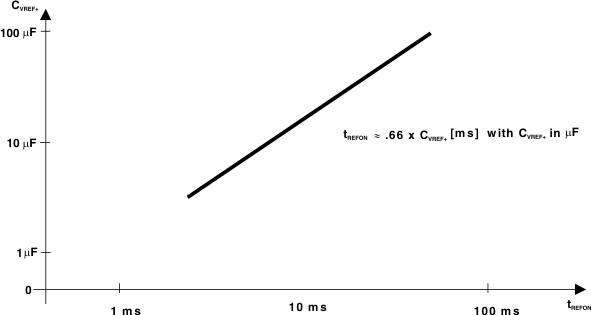Figure 4-39 Typical Settling Time of Internal Reference tREFON vs External Capacitor on VREF+Figure 4-40 Supply Voltage and Reference Voltage Design VREF-/VeREF- External Supply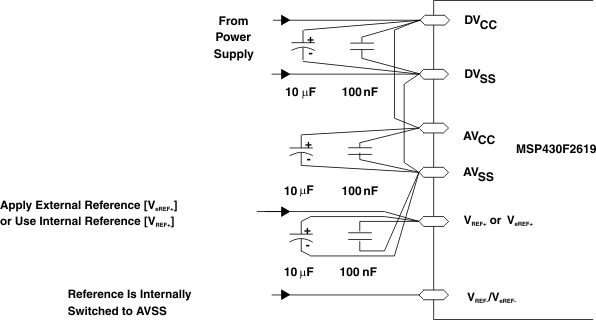Figure 4-41 Supply Voltage and Reference Voltage Design VREF-/VeREF- = AVSS, Internally Connected

### 4.50 12-Bit ADC Timing Parameters – Electrical Characteristics

over recommended ranges of supply voltage and operating free-air temperature (unless otherwise noted)
PARAMETER TEST CONDITIONS MIN TYP MAX UNIT
ƒADC12CLK ADC12 input clock frequency For specified performance of ADC12 linearity parameters, VCC = 2.2 V or 3 V 0.45 5 6.3 MHz
3.7 5 6.3 MHz
tCONVERT Conversion time ADC12 built-in oscillator, CVREF+ ≥ 5 μF,
ƒADC12OSC = 3.7 MHz to 6.3 MHz, VCC = 2.2 V or 3 V
2.06 3.51 μs
tSample(1) Sampling time RS = 400 Ω, RI = 1000 Ω, CI = 30 pF,
τ = [RS + RI] x CI (3)
VCC = 3 V 1220 ns
VCC = 2.2 V 1400
Limits verified by design.
The condition is that the error in a conversion started after tADC12ON is less than ±0.5 LSB. The reference and input signal are already settled.
Approximately ten Tau (τ) are needed to get an error of less than ±0.5 LSB: tSample = ln(2n+1) x (RS + RI) x CI+ 800 ns where n = ADC resolution = 12, RS = external source resistance.

### 4.51 12-Bit ADC Linearity Parameters – Electrical Characteristics

over recommended ranges of supply voltage and operating free-air temperature (unless otherwise noted)
PARAMETER TEST CONDITIONS MIN TYP MAX UNIT
EI Integral linearity error 1.4 V ≤ (VeREF+ - VREF-/VeREF-) min ≤ 1.6 V, VCC = 2.2 V or 3 V ±2 LSB
1.6 V < (VeREF+ - VREF-/VeREF-) min ≤ VAVCC, VCC = 2.2 V or 3 V ±1.7
ED Differential linearity error (VeREF+ - VREF-/VeREF-)min ≤ (VeREF+ - VREF-/VeREF-),
CVREF+ = 10 μF (tantalum) and 100 nF (ceramic),
VCC = 2.2 V or 3 V
±1 LSB
EO Offset error (VeREF+ - VREF-/VeREF-)min ≤ (VeREF+ - VREF-/VeREF-),
Internal impedance of source RS < 100 Ω,
CVREF+ = 10 μF (tantalum) and 100 nF (ceramic),
VCC = 2.2 V or 3 V
±2 ±4 LSB
EG Gain error (VeREF+ - VREF-/VeREF-)min ≤ (VeREF+ - VREF-/VeREF-),
CVREF+ = 10 μF (tantalum) and 100 nF (ceramic),
VCC = 2.2 V or 3 V
±1.1 ±2 LSB
ET Total unadjusted error (VeREF+ - VREF-/VeREF-)min ≤ (VeREF+ - VREF-/VeREF-),
CVREF+ = 10 μF (tantalum) and 100 nF (ceramic),
VCC = 2.2 V or 3 V
±2 ±5 LSB

### 4.52 12-Bit ADC Temperature Sensor and Built-In VMID – Electrical Characteristics

over recommended ranges of supply voltage and operating free-air temperature (unless otherwise noted)
PARAMETER TEST CONDITIONS MIN TYP MAX UNIT
ISENSOR Operating supply current into
AVCC terminal(2)
REFON = 0, INCH = 0Ah,
ADC12ON = 1, TA = 25°C,
VCC = 2.2 V
VCC = 2.2 V 40 120 μA
VCC = 3 V 60 160
VSensor(1) Sensor output voltage(3) ADC12ON = 1, INCH = 0Ah,
TA = 0°C,
VCC = 2.2 V or 3 V
986 mV
TCSENSOR(1) ADC12ON = 1, INCH = 0Ah, VCC = 2.2 V or 3 V 3.55 mV/°C
tSensor(sample)(1) Sample time required
if channel 10 is selected (4)
ADC12ON = 1, INCH = 0Ah,
Error of conversion result ≤ 1 LSB,
VCC = 2.2 V or 3 V
30 μs
IVMID Current into divider
at channel 11(5)
ADC12ON = 1, INCH = 0Bh VCC = 2.2 V NA μA
VCC = 3 V NA
VMID AVCC divider at channel 11 ADC12ON = 1, INCH = 0Bh,
VMID is ≉ 0.5 × VAVCC
VCC = 2.2 V 1.1 1.1±0.04 V
VCC = 3 V 1.5 1.5 ±0.04
tVMID(sample) Sample time required
if channel 11 is selected (6)
ADC12ON = 1, INCH = 0Bh,
Error of conversion result ≤ 1 LSB
VCC = 2.2 V 1400 ns
VCC = 3 V 1220
Limits characterized.
The sensor current ISENSOR is consumed if (ADC12ON = 1 and REFON = 1) or (ADC12ON = 1 and INCH = 0Ah and sample signal is high). When REFON = 1, ISENSOR is included in IREF+. When REFON = 0, ISENSOR applies during conversion of the temperature sensor input (INCH = 0Ah).
The temperature sensor offset can be as much as ±20°C. A single-point calibration is recommended to minimize the offset error of the built-in temperature sensor.
The typical equivalent impedance of the sensor is 51 kΩ. The sample time required includes the sensor-on time tSENSOR(on).
No additional current is needed. The VMID is used during sampling.
The on-time tVMID(on) is included in the sampling time tVMID(sample); no additional on time is needed.

### 4.53 12-Bit DAC Supply Specifications – Electrical Characteristics

over recommended ranges of supply voltage and operating free-air temperature (unless otherwise noted)
PARAMETER TEST CONDITIONS MIN TYP MAX UNIT
AVCC Supply voltage range AVCC = DVCC, AVSS = DVSS = 0 V 2.2 3.6 V
IDD Supply current,
single DAC channel(1)(2)
DAC12AMPx = 2, DAC12IR = 0,
DAC12_xDAT = 0x0800,
VCC = 2.2 V or 3 V
TA = -55°C to 85°C 50 110 μA
TA = 105°C 69 150
DAC12AMPx = 2, DAC12IR = 1, DAC12_xDAT = 0x0800,
VeREF+ = VREF+ = AVCC, VCC = 2.2 V or 3 V
50 130
DAC12AMPx = 5, DAC12IR = 1, DAC12_xDAT = 0x0800,
VeREF+ = VREF+ = AVCC, VCC = 2.2 V or 3 V
200 440
DAC12AMPx = 7, DAC12IR = 1, DAC12_xDAT = 0x0800,
VeREF+ = VREF+ = AVCC, VCC = 2.2 V or 3 V
700 1500
PSSR Power-supply rejection ratio(3)(4) DAC12_xDAT = 800h, VREF = 1.5 V,
ΔAVCC = 100 mV, VCC = 2.2 V or 3 V
70 dB
DAC12_xDAT = 800h, VREF = 1.5 V or 2.5 V,
ΔAVCC = 100 mV, VCC = 2.2 V or 3 V
70
No load at the output pin, DAC12_0 or DAC12_1, assuming that the control bits for the shared pins are set properly.
Current into reference terminals not included. If DAC12IR = 1 current flows through the input divider; see Section 4.57.
PSRR = 20 × log\{ΔAVCC/ΔVDAC12_xOUT\}
VREF is applied externally. The internal reference is not used.

### 4.54 12-Bit DAC Linearity Parameters – Electrical Characteristics

over recommended ranges of supply voltage and operating free-air temperature (unless otherwise noted)
PARAMETER TEST CONDITIONS MIN TYP MAX UNIT
Resolution 12-bit monotonic 12 bits
INL Integral nonlinearity(1) VREF = 1.5 V, DAC12AMPx = 7,
DAC12IR = 1, VCC = 2.2 V or 3 V
±2 ±8 LSB
VREF = 2.5 V, DAC12AMPx = 7,
DAC12IR = 1, VCC = 2.2 V or 3 V
±2 ±8
DNL Differential nonlinearity(1) VREF = 1.5 V, DAC12AMPx = 7,
DAC12IR = 1, VCC = 2.2 V or 3 V
±0.4 ±1 LSB
VREF = 2.5 V, DAC12AMPx = 7,
DAC12IR = 1, VCC = 2.2 V or 3 V
±0.4 ±1
EO Offset voltage without calibration(1)(2) VREF = 1.5 V, DAC12AMPx = 7,
DAC12IR = 1, VCC = 2.2 V or 3 V
±21 LSB
VREF = 2.5 V, DAC12AMPx = 7,
DAC12IR = 1, VCC = 2.2 V or 3 V
±21
Offset voltage with calibration(1)(2) VREF = 1.5 V, DAC12AMPx = 7,
DAC12IR = 1, VCC = 2.2 V or 3 V
±3.5
VREF = 2.5 V, DAC12AMPx = 7,
DAC12IR = 1, VCC = 2.2 V or 3 V
±3.5
dE(O)/dT Offset error temperature coefficient(1) 30 µV/°C
EG Gain error(1) VREF = 1.5 V, VCC = 2.2 V or 3 V ±3.5 LSB
VREF = 2.5 V, VCC = 2.2 V or 3 V ±3.5
dE(G)/dT Gain temperature coefficient(1) 10 ppm of
FSR/°C
tOffset_Cal Time for offset calibration(3) DAC12AMPx = 2, VCC = 2.2 V or 3 V 100 LSB
DAC12AMPx = 3, 5, VCC = 2.2 V or 3 V 32
DAC12AMPx = 4, 6, 7, VCC = 2.2 V or 3 V 6
Parameters calculated from the best-fit curve from 0x0A to 0xFFF. The best-fit curve method is used to deliver coefficients “a” and “b” of the first-order equation: y = a + b × x. VDAC12_xOUT = EO + (1 + EG) × (VeREF+/4095) × DAC12_xDAT, DAC12IR = 1.
The offset calibration works on the output operational amplifier. Offset calibration is triggered setting bit DAC12CALON.
The offset calibration can be done if DAC12AMPx = \{2, 3, 4, 5, 6, 7\}. The output operational amplifier is switched off with DAC12AMPx=\{0, 1\}.The DAC12 module should be configured prior to initiating calibration. Port activity during calibration may affect accuracy and is not recommended.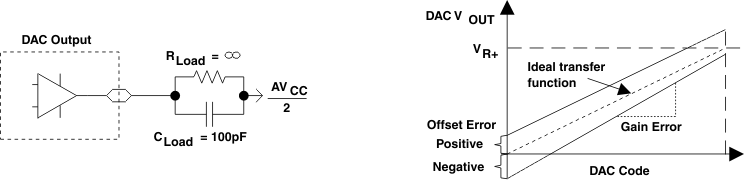Figure 4-42 Linearity Test Load Conditions and Gain/Offset Definition

### 4.55 Typical Characteristics - 12-Bit DAC Linearity Specifications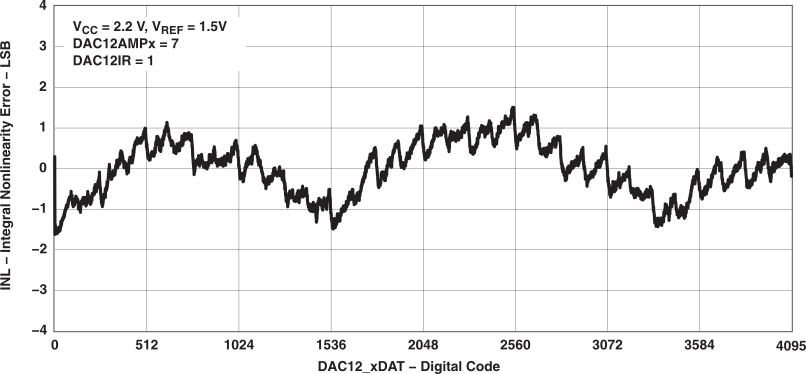Figure 4-43 Typical INL Error vs Digital Input Data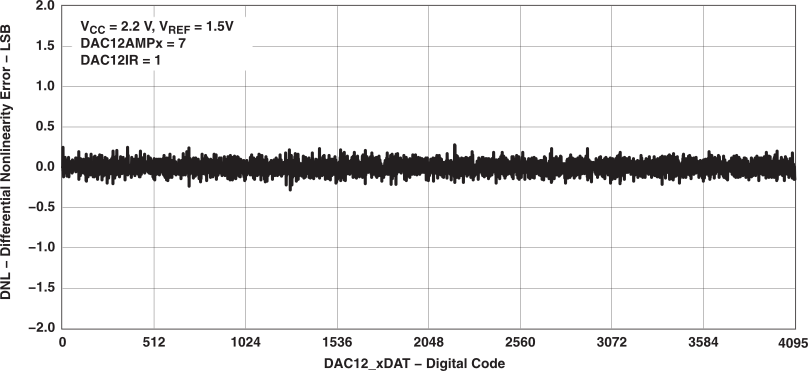Figure 4-44 Typical DNL Error vs Digital Input Data

### 4.56 12-Bit DAC Output Specifications – Electrical Characteristics

over recommended ranges of supply voltage and operating free-air temperature (unless otherwise noted)
PARAMETER TEST CONDITIONS MIN TYP MAX UNIT
VO Output voltage range(1)
(see Figure 4-45)
No Load, VeREF+ = AVCC, DAC12_xDAT = 0h,
DAC12IR = 1, DAC12AMPx = 7VCC = 2.2 V or 3 V
0 0.005 V
No Load, VeREF+ = AVCC, DAC12_xDAT = 0FFFh,
DAC12IR = 1, DAC12AMPx = 7VCC = 2.2 V or 3 V
AVCC - 0.05 AVCC
RLoad = 3 kΩ, VeREF+ = AVCC, DAC12_xDAT = 0h,
DAC12IR = 1, DAC12AMPx = 7, VCC = 2.2 V or 3 V
0 0.1
RLoad = 3 kΩ, VeREF+ = AVCC, DAC12_xDAT = 0FFFh,
DAC12IR = 1, DAC12AMPx = 7, VCC = 2.2 V or 3 V
AVCC - 0.13 AVCC
CL(DAC12) Max DAC12 load capacitance VCC = 2.2 V or 3 V 100 pF
IL(DAC12) Max DAC12 load current VCC = 2.2 V –0.5 0.5 mA
VCC = 3 V –1 1
RO/P(DAC12) Output resistance
(see Figure 4-45)
RLoad = 3 kΩ, VO/P(DAC12) = 0 V, DAC12AMPx = 7,
DAC12_xDAT = 0h, VCC = 2.2 V or 3 V
150 250 Ω
RLoad = 3 kΩ, VO/P(DAC12) = 0 V, DAC12AMPx = 7,
DAC12_xDAT = 0FFFh, VCC = 2.2 V or 3 V
150 250
RLoad = 3 kΩ, 0.3 V ≤ VO/P(DAC12) ≤ AVCC - 0.3 V,
DAC12AMPx = 7, VCC = 2.2 V or 3 V
1 4
Data is valid after the offset calibration of the output amplifier.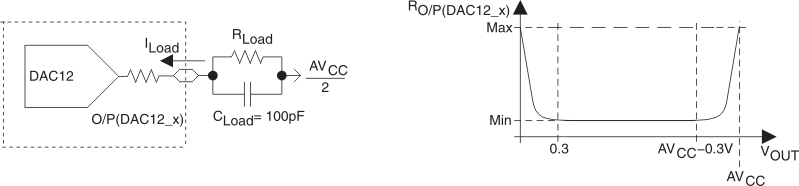Figure 4-45 DAC12_x Output Resistance Tests

### 4.57 12-Bit DAC Reference Input Specifications – Electrical Characteristics

over recommended ranges of supply voltage and operating free-air temperature (unless otherwise noted)
PARAMETER TEST CONDITIONS MIN TYP MAX UNIT
VeREF+ Reference input voltage range DAC12IR = 0(1)(2), VCC = 2.2 V or 3 V AVCC/3 AVCC + 0.2 V
DAC12IR = 1(3)(4), VCC = 2.2 V or 3 V AVCC AVCC + 0.2
Ri(VREF+),
Ri(VeREF+)
Reference input resistance DAC12_0 IR = DAC12_1 IR = 0,
VCC = 2.2 V or 3 V
20
DAC12_0 IR = 1, DAC12_1 IR = 0,
VCC = 2.2 V or 3 V
40 48 56
DAC12_0 IR = 0, DAC12_1 IR = 1,
VCC = 2.2 V or 3 V
20 24 28
DAC12_0 IR = 0, DAC12_1 IR = 1
DAC12_0 SREFx = DAC12_1 SREFx(5),
VCC = 2.2 V or 3 V
For a full-scale output, the reference input voltage can be as high as 1/3 of the maximum output voltage swing (AVCC).
The maximum voltage applied at reference input voltage terminal VeREF+ = [AVCC - VE(O)] / [3 x (1 + EG)].
For a full-scale output, the reference input voltage can be as high as the maximum output voltage swing (AVCC).
The maximum voltage applied at reference input voltage terminal VeREF+ = [AVCC - VE(O)] / (1 + EG).
When DAC12IR = 1 and DAC12SREFx = 0 or 1 for both channels, the reference input resistive dividers for each DAC are in parallel reducing the reference input resistance.

### 4.58 12-Bit DAC Dynamic Specifications, VREF = VCC, DAC12IR = 1 – Electrical Characteristics

over recommended ranges of supply voltage and operating free-air temperature (unless otherwise noted)
PARAMETER TEST CONDITIONS MIN TYP MAX UNIT
tON SR DAC12_xDAT = 800h,
ErrorV(O) < ±0.5 LSB(1) (see Figure 4-46), VCC = 2.2 V or 3 V
DAC12AMPx = 0 → \{2, 3, 4\} 60 120 μs
DAC12AMPx = 0 → \{5, 6\} 15 30
DAC12AMPx = 0 → 7 6 12
tS(FS) Settling time, full scale DAC12_xDAT =
80h → F7Fh → 80h, VCC = 2.2 V or 3 V
DAC12AMPx = 2 100 200 μs
DAC12AMPx = 3, 5 40 80
DAC12AMPx = 4, 6, 7 15 30
tS(C-C) Settling time, code to code DAC12_xDAT =
3F8h → 408h → 3F8h, VCC = 2.2 V or 3 V
DAC12AMPx = 2 5 μs
DAC12AMPx = 3, 5 2
DAC12AMPx = 4, 6, 7 1
SR Slew rate(2) DAC12_xDAT =
80h → F7Fh → 80h, VCC = 2.2 V or 3 V
DAC12AMPx = 2 0.05 0.12 V/μs
DAC12AMPx = 3, 5 0.35 0.7
DAC12AMPx = 4, 6, 7 1.5 2.7
Glitch energy, full scale DAC12_xDAT =
80h → F7Fh → 80h, VCC = 2.2 V or 3 V
DAC12AMPx = 2 600 nV-s
DAC12AMPx = 3, 5 150
DAC12AMPx = 4, 6, 7 30
BW-3dB 3-dB bandwidth,
VDC = 1.5 V,
VAC = 0.1 VPP
(see Figure 4-48)
DAC12AMPx = \{2, 3, 4\}, DAC12SREFx = 2,
DAC12IR = 1, DAC12_xDAT = 800h, VCC = 2.2 V or 3 V
40 kHz
DAC12AMPx = \{5, 6\}, DAC12SREFx = 2,
DAC12IR = 1, DAC12_xDAT = 800h, VCC = 2.2 V or 3 V
180
DAC12AMPx = 7, DAC12SREFx = 2,
DAC12IR = 1, DAC12_xDAT = 800h, VCC = 2.2 V or 3 V
550
Channel-to-channel crosstalk(3)
(see Figure 4-49)
DAC12_1DAT = 80h ↔ F7Fh, RLoad = 3 kΩ,
ƒDAC12_1OUT = 10 kHz, Duty cycle = 50%, VCC = 2.2 V or 3 V
–80 dB
DAC12_0DAT = 80h ↔ F7Fh, RLoad = 3 kΩ,
ƒDAC12_0OUT = 10 kHz, Duty cycle = 50%, VCC = 2.2 V or 3 V
–80
RLoad and CLoad are connected to AVSS (not AVCC/2) in Figure 4-46.
Slew rate applies to output voltage steps ≥ 200 mV.Figure 4-46 Settling Time and Glitch Energy TestingFigure 4-47 Slew Rate Testing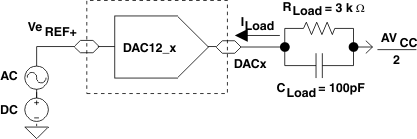Figure 4-48 Test Conditions for 3-dB Bandwidth Specification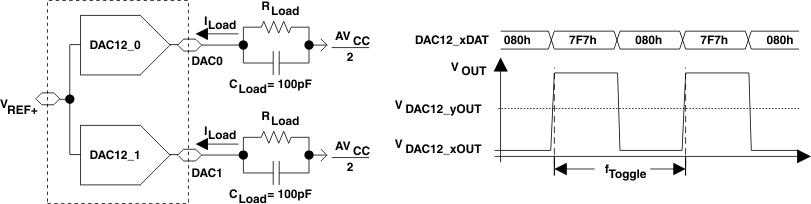Figure 4-49 Crosstalk Test Conditions

### 4.59 Flash Memory – Electrical Characteristics

over recommended ranges of supply voltage and operating free-air temperature (unless otherwise noted)
PARAMETER TEST CONDITIONS MIN TYP MAX UNIT
VCC(PGM/ERASE) Program and erase supply voltage 2.2 3.6 V
ƒFTG Flash timing generator frequency 257 476 kHz
IPGM Supply current from VCC during program VCC = 2.2 or 3.6 V 3 5 mA
IERASE Supply current from VCC during erase VCC = 2.2 or 3.6 V 3 7 mA
tCPT Cumulative program time See (1), VCC = 2.2 or 3.6 V 10 ms
tCMErase Cumulative mass erase time VCC = 2.2 or 3.6 V 20 ms
Program/Erase endurance 104 105 cycles
tRetention Data retention duration TJ = 25°C 100 years
tWord Word or byte program time See(2) 35 tFTG
tBlock, 0 Block program time for 1st byte or word See(2)  30 tFTG
tBlock, 1-63 Block program time for each additional byte or word See(2) 21 tFTG
tBlock, End Block program end-sequence wait time See(2) 6 tFTG
tMass Erase Mass erase time See(2) 10593 tFTG
tSeg Erase Segment erase time See(2) 4819 tFTG
The cumulative program time must not be exceeded when writing to a 64-byte flash block. This parameter applies to all programming methods: individual word/byte write and block write modes.
These values are hardwired into the Flash Controller's state machine (tFTG = 1/ƒFTG).Figure 4-50 Flash Data Retention vs Junction Temperature

### 4.60 RAM – Electrical Characteristics

over recommended ranges of supply voltage and operating free-air temperature (unless otherwise noted)
PARAMETER TEST CONDITIONS MIN MAX UNIT
V(RAMh) RAM retention supply voltage (1) CPU halted 1.6 V
This parameter defines the minimum supply voltage VCC when the data in RAM remains unchanged. No program execution should happen during this supply voltage condition.

### 4.61 JTAG and Spy-Bi-Wire Interface – Electrical Characteristics

over recommended ranges of supply voltage and operating free-air temperature (unless otherwise noted)
PARAMETER TEST CONDITIONS MIN TYP MAX UNIT
ƒTCK TCK input frequency See (1) VCC = 2.2 V 0 5 MHz
VCC = 3 V 0 10
RInternal Internal pulldown resistance on TEST See (2), VCC = 2.2 V or 3 V 25 60 90
ƒTCK may be restricted to meet the timing requirements of the module selected.
TMS, TDI/TCLK, and TCK pullup resistors are implemented in all versions.

### 4.62 JTAG Fuse(1) – Electrical Characteristics

over recommended ranges of supply voltage and operating free-air temperature (unless otherwise noted)
PARAMETER TEST CONDITIONS MIN MAX UNIT
VCC(FB) Supply voltage during fuse-blow condition TA = 25°C 2.5 V
VFB Voltage level on TEST for fuse blow 6 7 V
IFB Supply current into TEST during fuse blow 100 mA
tFB Time to blow fuse 1 ms
Once the fuse is blown, no further access to the MSP430 JTAG/Test and emulation features is possible. The JTAG block is switched to bypass mode.